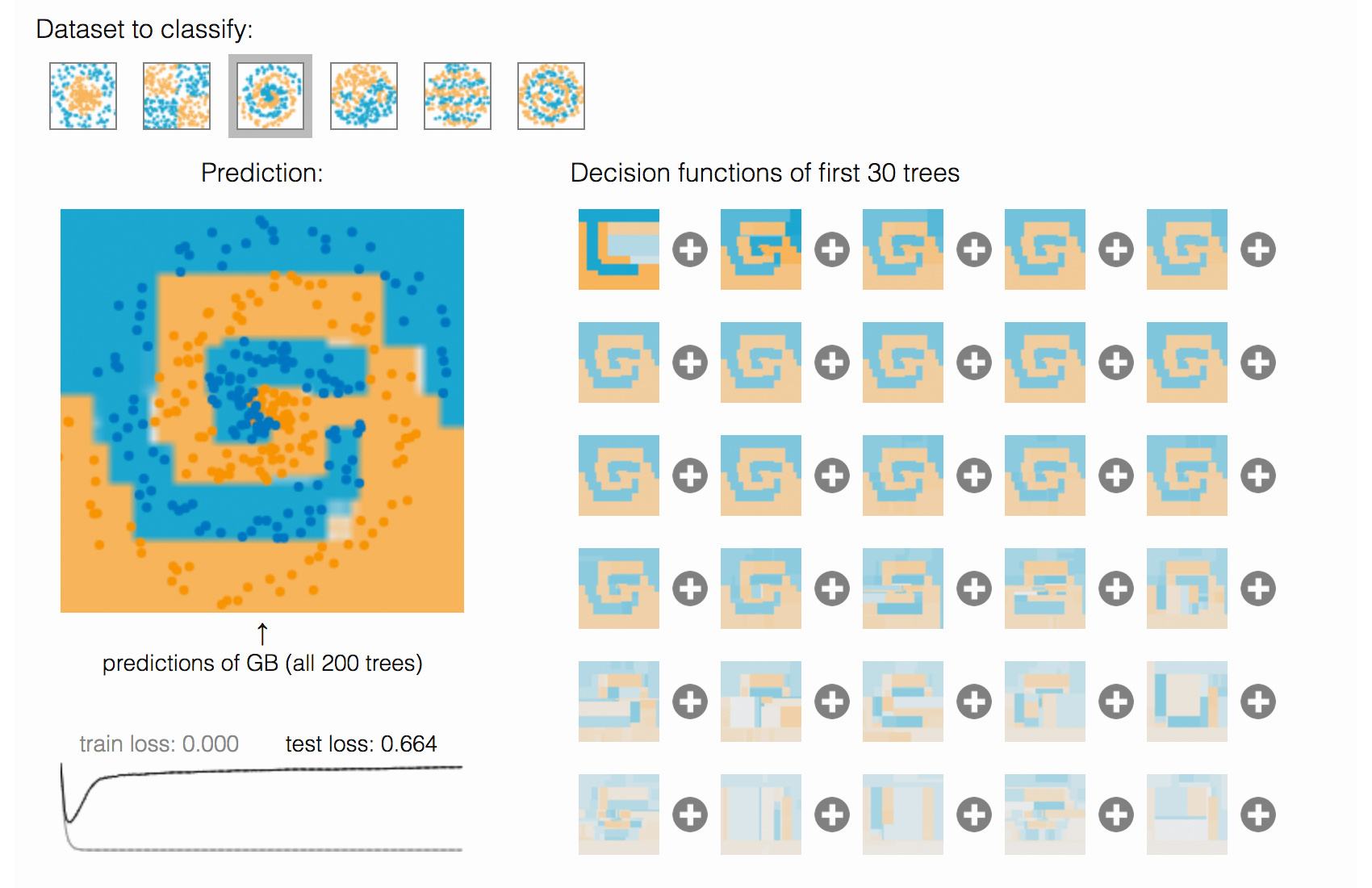## 集成学习和随机森林方法¶

• 集成
• Bootstraping
• Bagging
• 随机森林
• 特征重要性

### 集成¶

$$\mu = \sum_{i=m}^{N}{N\choose i}p^i(1-p)^{N-i}$$

• $N$ 为陪审员总数。
• $m$ 是构成多数的最小值，即 $m=\operatorname{floor}(N / 2)+1$。
• ${N \choose i}$ 是组合数。
• $p$ 为评审员做出正确决策的概率。
• $\mu$ 是整个评审团做出正确决策的概率。

### Bootstrapping¶

Leo Breiman 于 1994 年提出的 Bagging（又称 Bootstrap Aggregation，引导聚集）是最基本的集成技术之一。Bagging 基于统计学中的 Bootstraping（自助法），该方法令复杂模型的统计评估变得更加可行。

Bootstrap 方法的流程如下：假设有尺寸为 N 的样本 X，从该样本中有放回地随机抽取 N 个样本，以创建一个新样本。换句话说，从尺寸为 N 的原样本中随机选择一个元素，并重复此过程 N 次。选中所有元素的可能性是一样的，因此每个元素被抽中的概率均为 $\frac{1}{N}$。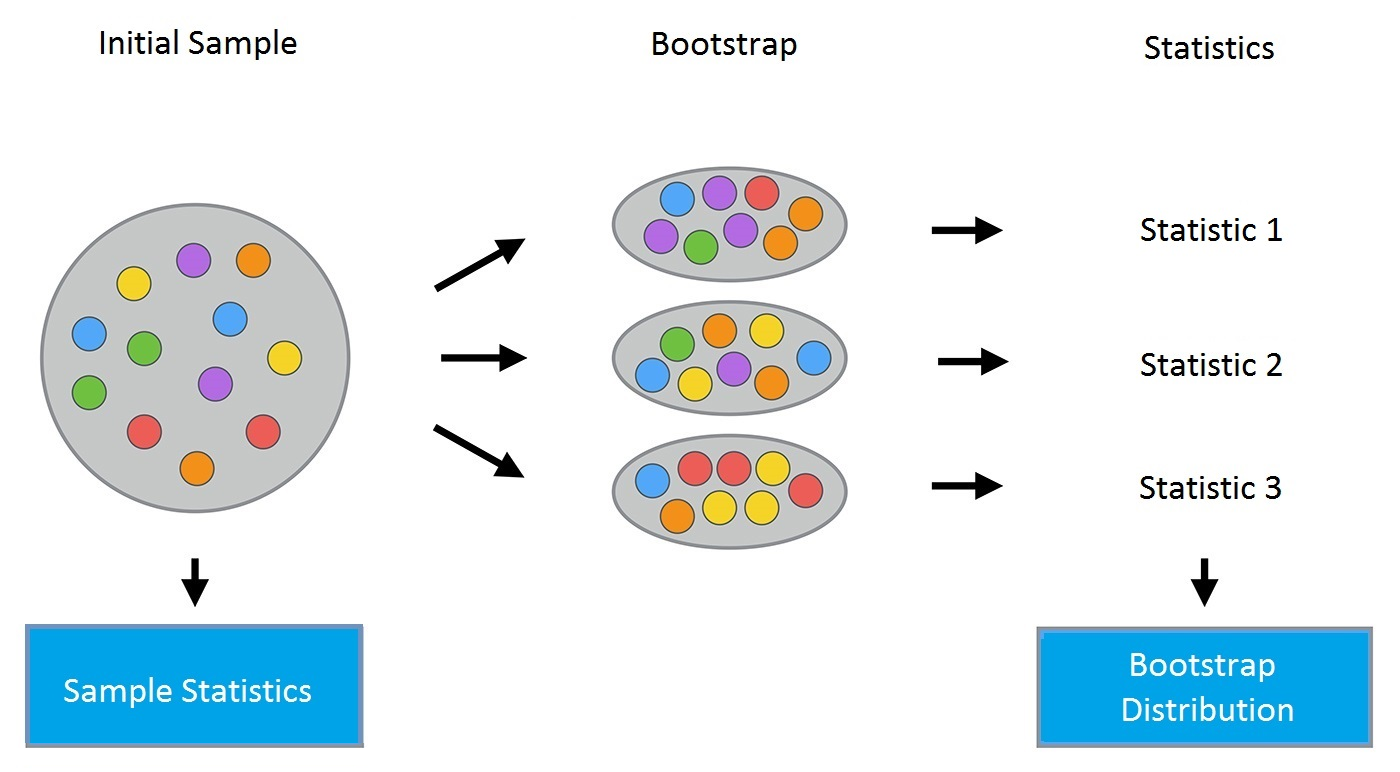In :
import warnings

import numpy as np
import pandas as pd
import seaborn as sns
from matplotlib import pyplot as plt

plt.style.use('ggplot')
plt.rcParams['figure.figsize'] = 10, 6
%matplotlib inline
warnings.filterwarnings('ignore')

In :
telecom_data = pd.read_csv(
'../../data/telecom_churn.csv')

fig = sns.kdeplot(telecom_data[telecom_data['Churn']
== False]['Customer service calls'], label='Loyal')
fig = sns.kdeplot(telecom_data[telecom_data['Churn']
== True]['Customer service calls'], label='Churn')
fig.set(xlabel='Number of calls', ylabel='Density')
plt.show()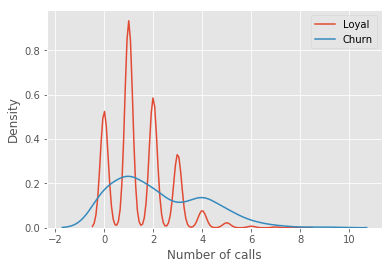In :
def get_bootstrap_samples(data, n_samples):
indices = np.random.randint(0, len(data), (n_samples, len(data)))
samples = data[indices]
return samples

def stat_intervals(stat, alpha):
boundaries = np.percentile(
stat, [100 * alpha / 2., 100 * (1 - alpha / 2.)])
return boundaries


In :
loyal_calls = telecom_data.loc[telecom_data['Churn'] == False,
'Customer service calls'].values
churn_calls = telecom_data.loc[telecom_data['Churn'] == True,
'Customer service calls'].values


In :
np.random.seed(0)


In :
loyal_mean_scores = [np.mean(sample)
for sample in get_bootstrap_samples(loyal_calls, 1000)]
churn_mean_scores = [np.mean(sample)
for sample in get_bootstrap_samples(churn_calls, 1000)]


In :
print("Service calls from loyal: mean interval",
stat_intervals(loyal_mean_scores, 0.05))
print("Service calls from churn: mean interval",
stat_intervals(churn_mean_scores, 0.05))

Service calls from loyal: mean interval [1.4077193  1.49473684]
Service calls from churn: mean interval [2.0621118  2.39761905]


### Bagging¶

$$a(x) = \frac{1}{M}\sum_{i = 1}^M a_i(x).$$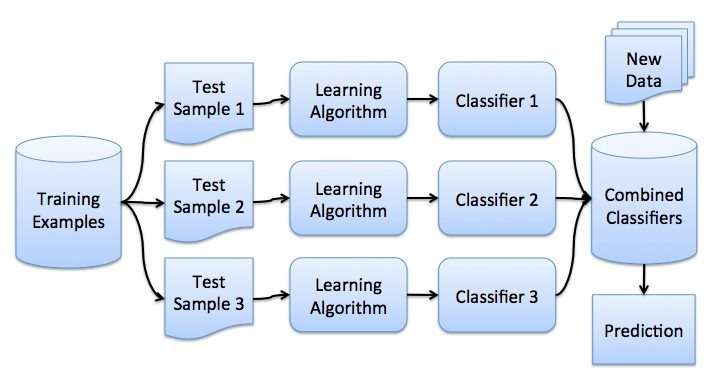$$\mathrm{Bias}\left(\hat{f}\right)^2 + \mathrm{Var}\left(\hat{f}\right) + \sigma^2$$

Bagging 通过在不同数据集上训练模型的方式来降低分类器的方差。换句话说，Bagging 可以预防过拟合。由于来自不同训练数据集上的模型各不相同，它们的误差在投票过程中会相互抵消，所以 Bagging 是有效的。此外，某些 Bootstrap 训练样本很可能会忽略异常值。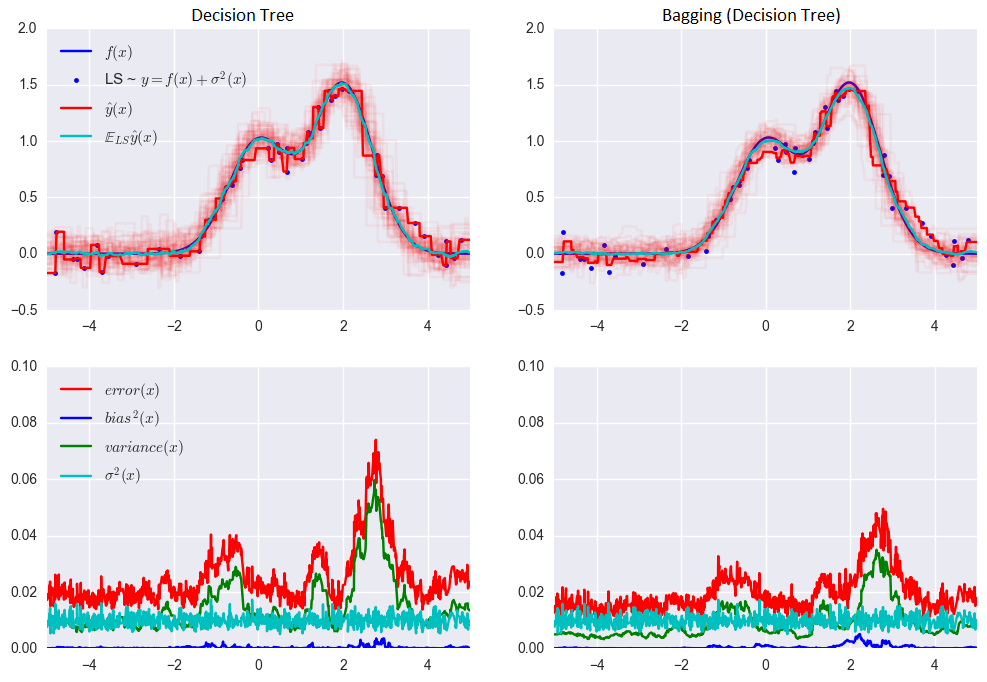$$0.0255 (\mathrm{Err}) = 0.0003 (\mathrm{Bias}^2) + 0.0152 (\mathrm{Var}) + 0.0098 (\sigma^2)$$

Bagging 的误差：

$$0.0196 \, (\mathrm{Err}) = 0.0004 \, (\mathrm{Bias}^2) + 0.0092 \, (\mathrm{Var}) + 0.0098 \, (\sigma^2)$$

### 袋外误差（OOB Error）¶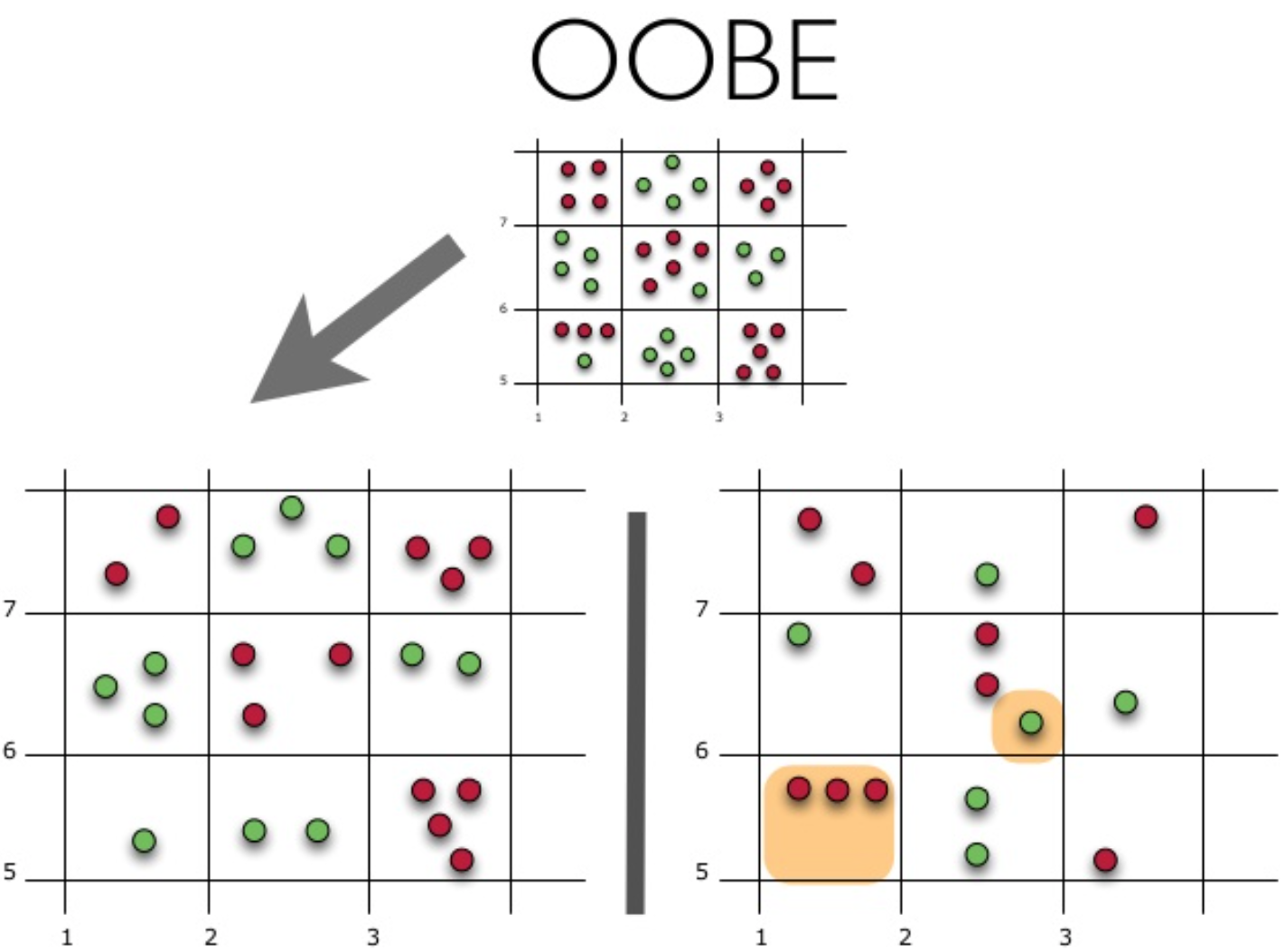### 随机森林¶

• 设样本数等于 n，特征维度数等于 d。
• 选择模型的数目 M。
• 对于每个模型 m，选择特征数 dm<d。所有模型使用相同的 dm 值。
• 对每个模型 m，通过在整个 d 特征集合上随机选择 dm 个特征创建一个训练集。
• 训练每个模型。
• 通过组合 M 个模型的结果，将生成的整体模型应用于新数据中，应用时可以基于多数投票算法（majority voting）或后验概率聚合（aggregation of the posterior probabilities）。

#### 随机森林的算法¶

• 对每个 $k = 1, \dots, N$，生成 Bootstrap 样本 $X_k$。
• 在样本 $X_k$ 上创建一棵决策树 $b_k$：
• 根据给定的标准选择最佳的特征维度。根据该特征分割样本以创建树的新层。重复这一流程，直到样本用尽。
• 创建树，直到任何叶节点包含的实例不超过 $n_\text{min}$ 个，或者达到特定深度。
• 每次分割，首先从 $d$ 个原始特征中随机选择 $m$ 个特征，接着只在该子集上搜索最佳分割。

$$a(x) = \frac{1}{N}\sum_{k = 1}^N b_k(x)$$

#### 随机森林与 Bagging 和决策树的比较¶

In :
from __future__ import division, print_function

# Disable warnings in Anaconda
import warnings

import numpy as np
import seaborn as sns
from matplotlib import pyplot as plt
from sklearn.datasets import make_circles
from sklearn.ensemble import (BaggingClassifier, BaggingRegressor,
RandomForestClassifier, RandomForestRegressor)
from sklearn.model_selection import train_test_split
from sklearn.tree import DecisionTreeClassifier, DecisionTreeRegressor

warnings.filterwarnings('ignore')

%matplotlib inline
plt.style.use('ggplot')
plt.rcParams['figure.figsize'] = 10, 6

n_train = 150
n_test = 1000
noise = 0.1


In :
def f(x):
x = x.ravel()
return np.exp(-x ** 2) + 1.5 * np.exp(-(x - 2) ** 2)

def generate(n_samples, noise):
X = np.random.rand(n_samples) * 10 - 5
X = np.sort(X).ravel()
y = np.exp(-X ** 2) + 1.5 * np.exp(-(X - 2) ** 2)\
+ np.random.normal(0.0, noise, n_samples)
X = X.reshape((n_samples, 1))

return X, y

X_train, y_train = generate(n_samples=n_train, noise=noise)
X_test, y_test = generate(n_samples=n_test, noise=noise)


In :
dtree = DecisionTreeRegressor().fit(X_train, y_train)
d_predict = dtree.predict(X_test)

plt.figure(figsize=(10, 6))
plt.plot(X_test, f(X_test), "b")
plt.scatter(X_train, y_train, c="b", s=20)
plt.plot(X_test, d_predict, "g", lw=2)
plt.xlim([-5, 5])
plt.title("Decision tree, MSE = %.2f"
% np.sum((y_test - d_predict) ** 2))

Out:
Text(0.5, 1.0, 'Decision tree, MSE = 20.09')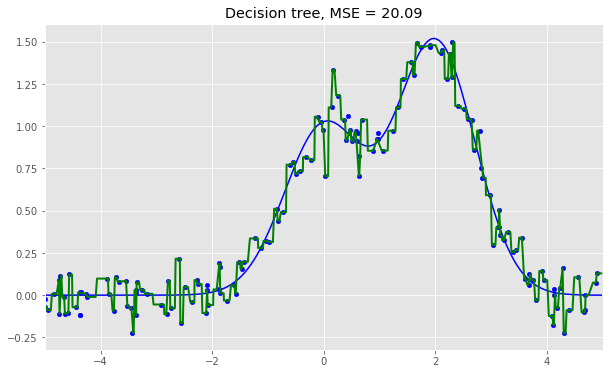In :
bdt = BaggingRegressor(DecisionTreeRegressor()).fit(X_train, y_train)
bdt_predict = bdt.predict(X_test)

plt.figure(figsize=(10, 6))
plt.plot(X_test, f(X_test), "b")
plt.scatter(X_train, y_train, c="b", s=20)
plt.plot(X_test, bdt_predict, "y", lw=2)
plt.xlim([-5, 5])
plt.title("Bagging for decision trees, MSE = %.2f" %
np.sum((y_test - bdt_predict) ** 2))

Out:
Text(0.5, 1.0, 'Bagging for decision trees, MSE = 15.24')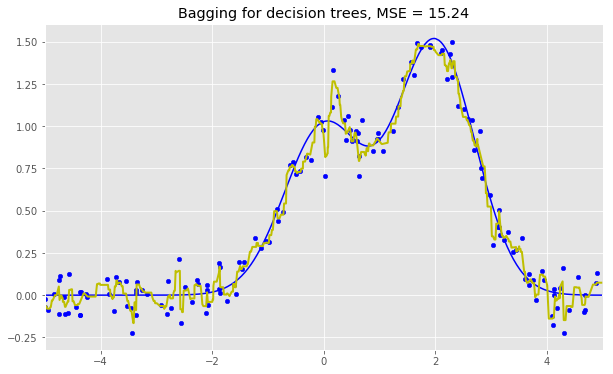In :
rf = RandomForestRegressor(n_estimators=10).fit(X_train, y_train)
rf_predict = rf.predict(X_test)

plt.figure(figsize=(10, 6))
plt.plot(X_test, f(X_test), "b")
plt.scatter(X_train, y_train, c="b", s=20)
plt.plot(X_test, rf_predict, "r", lw=2)
plt.xlim([-5, 5])
plt.title("Random forest, MSE = %.2f" % np.sum((y_test - rf_predict) ** 2))

Out:
Text(0.5, 1.0, 'Random forest, MSE = 15.71')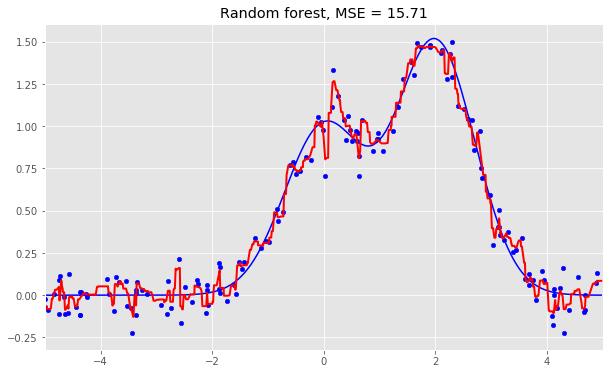In :
np.random.seed(42)
X, y = make_circles(n_samples=500, factor=0.1, noise=0.35, random_state=42)
X_train_circles, X_test_circles, y_train_circles, y_test_circles = train_test_split(
X, y, test_size=0.2)

dtree = DecisionTreeClassifier(random_state=42)
dtree.fit(X_train_circles, y_train_circles)

x_range = np.linspace(X.min(), X.max(), 100)
xx1, xx2 = np.meshgrid(x_range, x_range)
y_hat = dtree.predict(np.c_[xx1.ravel(), xx2.ravel()])
y_hat = y_hat.reshape(xx1.shape)
plt.contourf(xx1, xx2, y_hat, alpha=0.2)
plt.scatter(X[:, 0], X[:, 1], c=y, cmap='autumn')
plt.title("Decision tree")
plt.show()

b_dtree = BaggingClassifier(
DecisionTreeClassifier(), n_estimators=300, random_state=42)
b_dtree.fit(X_train_circles, y_train_circles)

x_range = np.linspace(X.min(), X.max(), 100)
xx1, xx2 = np.meshgrid(x_range, x_range)
y_hat = b_dtree.predict(np.c_[xx1.ravel(), xx2.ravel()])
y_hat = y_hat.reshape(xx1.shape)
plt.contourf(xx1, xx2, y_hat, alpha=0.2)
plt.scatter(X[:, 0], X[:, 1], c=y, cmap='autumn')
plt.title("Bagging (decision trees)")
plt.show()

rf = RandomForestClassifier(n_estimators=300, random_state=42)
rf.fit(X_train_circles, y_train_circles)

x_range = np.linspace(X.min(), X.max(), 100)
xx1, xx2 = np.meshgrid(x_range, x_range)
y_hat = rf.predict(np.c_[xx1.ravel(), xx2.ravel()])
y_hat = y_hat.reshape(xx1.shape)
plt.contourf(xx1, xx2, y_hat, alpha=0.2)
plt.scatter(X[:, 0], X[:, 1], c=y, cmap='autumn')
plt.title("Random forest")
plt.show()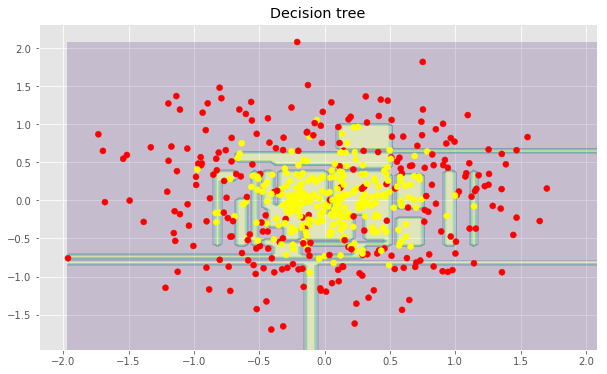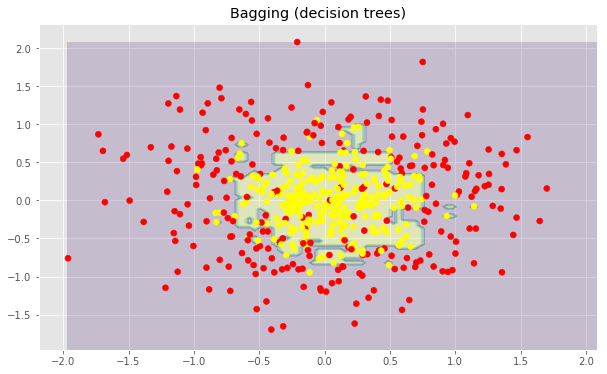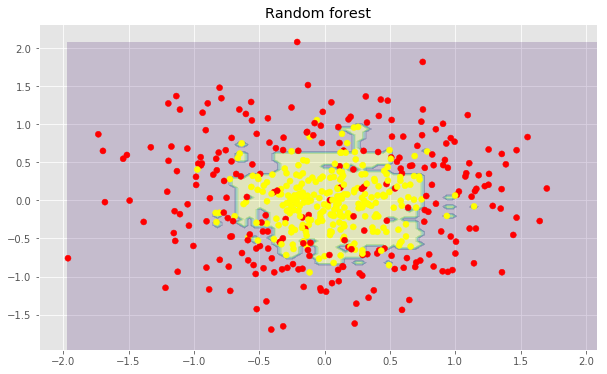#### 随机森林的参数¶

scikit-learn 库提供了 BaggingRegressorBaggingClassifier 类，其中在创建新模型时需要注意的一些参数如下：

• n_estimators 是随机森林中树的数量；
• criterion 是衡量分割质量的函数；
• max_features 是查找最佳分割时考虑的特征数；
• min_samples_leaf 是叶节点的最小样本数；
• max_depth 是树的最大深度。

#### 随机森林在现实问题中的应用¶

In :
import pandas as pd
from sklearn.metrics import accuracy_score
from sklearn.model_selection import (GridSearchCV, StratifiedKFold,
cross_val_score)


In :
df = pd.read_csv(
"../../data/telecom_churn.csv")


In :
cols = []
for i in df.columns:
if (df[i].dtype == "float64") or (df[i].dtype == 'int64'):
cols.append(i)


In :
X, y = df[cols].copy(), np.asarray(df["Churn"], dtype='int8')


In :
skf = StratifiedKFold(n_splits=5, shuffle=True, random_state=42)


In :
rfc = RandomForestClassifier(random_state=42, n_jobs=-1, oob_score=True)


In :
results = cross_val_score(rfc, X, y, cv=skf)


In :
print("CV accuracy score: {:.2f}%".format(results.mean()*100))

CV accuracy score: 91.48%


In :
skf = StratifiedKFold(n_splits=5, shuffle=True, random_state=42)


In :
train_acc = []
test_acc = []
temp_train_acc = []
temp_test_acc = []


In :
trees_grid = [5, 10, 15, 20, 30, 50, 75, 100]


In :
for ntrees in trees_grid:
rfc = RandomForestClassifier(
n_estimators=ntrees, random_state=42, n_jobs=-1, oob_score=True)
temp_train_acc = []
temp_test_acc = []
for train_index, test_index in skf.split(X, y):
X_train, X_test = X.iloc[train_index], X.iloc[test_index]
y_train, y_test = y[train_index], y[test_index]
rfc.fit(X_train, y_train)
temp_train_acc.append(rfc.score(X_train, y_train))
temp_test_acc.append(rfc.score(X_test, y_test))
train_acc.append(temp_train_acc)
test_acc.append(temp_test_acc)


In :
train_acc, test_acc = np.asarray(train_acc), np.asarray(test_acc)
print("Best accuracy on CV is {:.2f}% with {} trees".format(max(test_acc.mean(axis=1))*100,
trees_grid[np.argmax(test_acc.mean(axis=1))]))

Best accuracy on CV is 92.44% with 50 trees


In :
plt.style.use('ggplot')

fig, ax = plt.subplots(figsize=(8, 4))
ax.plot(trees_grid, train_acc.mean(axis=1),
alpha=0.5, color='blue', label='train')
ax.plot(trees_grid, test_acc.mean(axis=1), alpha=0.5, color='red', label='cv')
ax.fill_between(trees_grid, test_acc.mean(axis=1) - test_acc.std(axis=1),
test_acc.mean(axis=1) + test_acc.std(axis=1), color='#888888', alpha=0.4)
ax.fill_between(trees_grid, test_acc.mean(axis=1) - 2*test_acc.std(axis=1),
test_acc.mean(axis=1) + 2*test_acc.std(axis=1), color='#888888', alpha=0.2)
ax.legend(loc='best')
ax.set_ylim([0.88, 1.02])
ax.set_ylabel("Accuracy")
ax.set_xlabel("N_estimators")

Out:
Text(0.5, 0, 'N_estimators')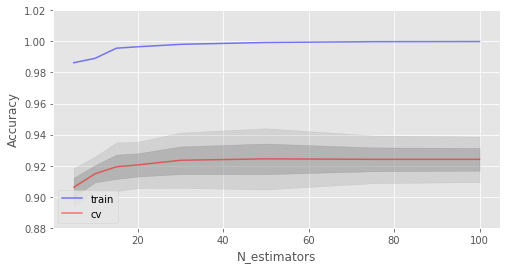In :
train_acc = []
test_acc = []
temp_train_acc = []
temp_test_acc = []
max_depth_grid = [3, 5, 7, 9, 11, 13, 15, 17, 20, 22, 24]


In :
for max_depth in max_depth_grid:
rfc = RandomForestClassifier(
n_estimators=100, random_state=42, n_jobs=-1, oob_score=True, max_depth=max_depth)
temp_train_acc = []
temp_test_acc = []
for train_index, test_index in skf.split(X, y):
X_train, X_test = X.iloc[train_index], X.iloc[test_index]
y_train, y_test = y[train_index], y[test_index]
rfc.fit(X_train, y_train)
temp_train_acc.append(rfc.score(X_train, y_train))
temp_test_acc.append(rfc.score(X_test, y_test))
train_acc.append(temp_train_acc)
test_acc.append(temp_test_acc)


In :
train_acc, test_acc = np.asarray(train_acc), np.asarray(test_acc)
print("Best accuracy on CV is {:.2f}% with {} max_depth".format(max(test_acc.mean(axis=1))*100,
max_depth_grid[np.argmax(test_acc.mean(axis=1))]))

fig, ax = plt.subplots(figsize=(8, 4))
ax.plot(max_depth_grid, train_acc.mean(axis=1),
alpha=0.5, color='blue', label='train')
ax.plot(max_depth_grid, test_acc.mean(axis=1),
alpha=0.5, color='red', label='cv')
ax.fill_between(max_depth_grid, test_acc.mean(axis=1) - test_acc.std(axis=1),
test_acc.mean(axis=1) + test_acc.std(axis=1), color='#888888', alpha=0.4)
ax.fill_between(max_depth_grid, test_acc.mean(axis=1) - 2*test_acc.std(axis=1),
test_acc.mean(axis=1) + 2*test_acc.std(axis=1), color='#888888', alpha=0.2)
ax.legend(loc='best')
ax.set_ylim([0.88, 1.02])
ax.set_ylabel("Accuracy")
ax.set_xlabel("Max_depth")

Best accuracy on CV is 92.68% with 17 max_depth

Out:
Text(0.5, 0, 'Max_depth')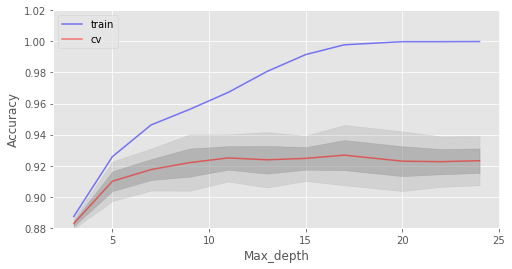max_depth 在模型中起到了正则化的作用，过拟合现象有所缓解，模型准确率提高了一些。

In :
train_acc = []
test_acc = []
temp_train_acc = []
temp_test_acc = []
min_samples_leaf_grid = [1, 3, 5, 7, 9, 11, 13, 15, 17, 20, 22, 24]


In :
for min_samples_leaf in min_samples_leaf_grid:
rfc = RandomForestClassifier(n_estimators=100, random_state=42, n_jobs=-1,
oob_score=True, min_samples_leaf=min_samples_leaf)
temp_train_acc = []
temp_test_acc = []
for train_index, test_index in skf.split(X, y):
X_train, X_test = X.iloc[train_index], X.iloc[test_index]
y_train, y_test = y[train_index], y[test_index]
rfc.fit(X_train, y_train)
temp_train_acc.append(rfc.score(X_train, y_train))
temp_test_acc.append(rfc.score(X_test, y_test))
train_acc.append(temp_train_acc)
test_acc.append(temp_test_acc)


In :
train_acc, test_acc = np.asarray(train_acc), np.asarray(test_acc)
print("Best accuracy on CV is {:.2f}% with {} min_samples_leaf".format(max(test_acc.mean(axis=1))*100,
min_samples_leaf_grid[np.argmax(test_acc.mean(axis=1))]))

fig, ax = plt.subplots(figsize=(8, 4))
ax.plot(min_samples_leaf_grid, train_acc.mean(
axis=1), alpha=0.5, color='blue', label='train')
ax.plot(min_samples_leaf_grid, test_acc.mean(
axis=1), alpha=0.5, color='red', label='cv')
ax.fill_between(min_samples_leaf_grid, test_acc.mean(axis=1) - test_acc.std(axis=1),
test_acc.mean(axis=1) + test_acc.std(axis=1), color='#888888', alpha=0.4)
ax.fill_between(min_samples_leaf_grid, test_acc.mean(axis=1) - 2*test_acc.std(axis=1),
test_acc.mean(axis=1) + 2*test_acc.std(axis=1), color='#888888', alpha=0.2)
ax.legend(loc='best')
ax.set_ylim([0.88, 1.02])
ax.set_ylabel("Accuracy")
ax.set_xlabel("Min_samples_leaf")

Best accuracy on CV is 92.41% with 3 min_samples_leaf

Out:
Text(0.5, 0, 'Min_samples_leaf')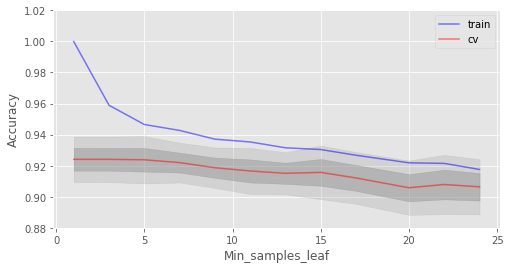In :
train_acc = []
test_acc = []
temp_train_acc = []
temp_test_acc = []
max_features_grid = [2, 4, 6, 8, 10, 12, 14, 16]


In :
for max_features in max_features_grid:
rfc = RandomForestClassifier(n_estimators=100, random_state=42, n_jobs=-1,
oob_score=True, max_features=max_features)
temp_train_acc = []
temp_test_acc = []
for train_index, test_index in skf.split(X, y):
X_train, X_test = X.iloc[train_index], X.iloc[test_index]
y_train, y_test = y[train_index], y[test_index]
rfc.fit(X_train, y_train)
temp_train_acc.append(rfc.score(X_train, y_train))
temp_test_acc.append(rfc.score(X_test, y_test))
train_acc.append(temp_train_acc)
test_acc.append(temp_test_acc)


In :
train_acc, test_acc = np.asarray(train_acc), np.asarray(test_acc)
print("Best accuracy on CV is {:.2f}% with {} max_features".format(max(test_acc.mean(axis=1))*100,
max_features_grid[np.argmax(test_acc.mean(axis=1))]))

fig, ax = plt.subplots(figsize=(8, 4))
ax.plot(max_features_grid, train_acc.mean(axis=1),
alpha=0.5, color='blue', label='train')
ax.plot(max_features_grid, test_acc.mean(axis=1),
alpha=0.5, color='red', label='cv')
ax.fill_between(max_features_grid, test_acc.mean(axis=1) - test_acc.std(axis=1),
test_acc.mean(axis=1) + test_acc.std(axis=1), color='#888888', alpha=0.4)
ax.fill_between(max_features_grid, test_acc.mean(axis=1) - 2*test_acc.std(axis=1),
test_acc.mean(axis=1) + 2*test_acc.std(axis=1), color='#888888', alpha=0.2)
ax.legend(loc='best')
ax.set_ylim([0.88, 1.02])
ax.set_ylabel("Accuracy")
ax.set_xlabel("Max_features")

Best accuracy on CV is 92.59% with 12 max_features

Out:
Text(0.5, 0, 'Max_features')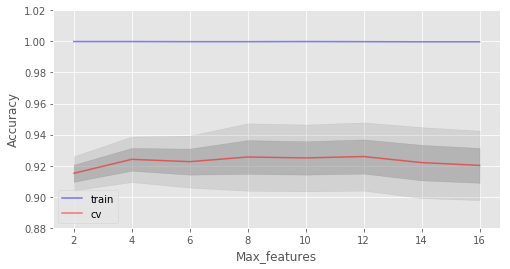In :
# 原实验搜索参数
# parameters = {'max_features': [4, 7, 10, 13], 'min_samples_leaf': [
#     1, 3, 5, 7], 'max_depth': [5, 10, 15, 20]}
# 为加速线上环境执行，优化搜索参数
parameters = {'max_features': [10, 13], 'min_samples_leaf': [1, 3], 'max_depth': [5, 10]}
rfc = RandomForestClassifier(n_estimators=100, random_state=42,
n_jobs=-1, oob_score=True)
gcv = GridSearchCV(rfc, parameters, n_jobs=-1, cv=skf, verbose=1)
gcv.fit(X, y)

Fitting 5 folds for each of 8 candidates, totalling 40 fits

[Parallel(n_jobs=-1)]: Using backend LokyBackend with 2 concurrent workers.
[Parallel(n_jobs=-1)]: Done  40 out of  40 | elapsed:   33.1s finished

Out:
GridSearchCV(cv=StratifiedKFold(n_splits=5, random_state=42, shuffle=True),
error_score='raise-deprecating',
estimator=RandomForestClassifier(bootstrap=True, class_weight=None, criterion='gini',
max_depth=None, max_features='auto', max_leaf_nodes=None,
min_impurity_decrease=0.0, min_impurity_split=None,
min_samples_leaf=1, min_samples_split=2,
min_weight_fraction_leaf=0.0, n_estimators=100, n_jobs=-1,
oob_score=True, random_state=42, verbose=0, warm_start=False),
fit_params=None, iid='warn', n_jobs=-1,
param_grid={'max_features': [10, 13], 'min_samples_leaf': [1, 3], 'max_depth': [5, 10]},
pre_dispatch='2*n_jobs', refit=True, return_train_score='warn',
scoring=None, verbose=1)

In :
gcv.best_estimator_, gcv.best_score_

Out:
(RandomForestClassifier(bootstrap=True, class_weight=None, criterion='gini',
max_depth=10, max_features=10, max_leaf_nodes=None,
min_impurity_decrease=0.0, min_impurity_split=None,
min_samples_leaf=1, min_samples_split=2,
min_weight_fraction_leaf=0.0, n_estimators=100, n_jobs=-1,
oob_score=True, random_state=42, verbose=0, warm_start=False),
0.9270927092709271)

#### 随机森林的方差和去相关¶

$$\mathrm{Var} f(x) = \rho(x)\sigma^2(x)$$
$$\rho(x) = \mathrm{Corr}\left[T(x_1,\Theta_1(Z)),T(x_2,\Theta_2(Z))\right],$$

• $\rho(x)$ 为任意两棵树之间的样本相关性；
• $\Theta_1(Z)$ 和 $\Theta_2(Z)$ 为在样本 $Z$ 的随机元素上，随机选择的一对树；
• $T(x,\Theta_i(Z))$ 为第 $i$ 个树分类器在输入向量 $x$ 上的输出；
• $\sigma^2(x)$ 为任何随机选择的树上的样本方差：$\sigma^2(x) = \mathrm{Var}\left[T(x,\Theta(X))\right]$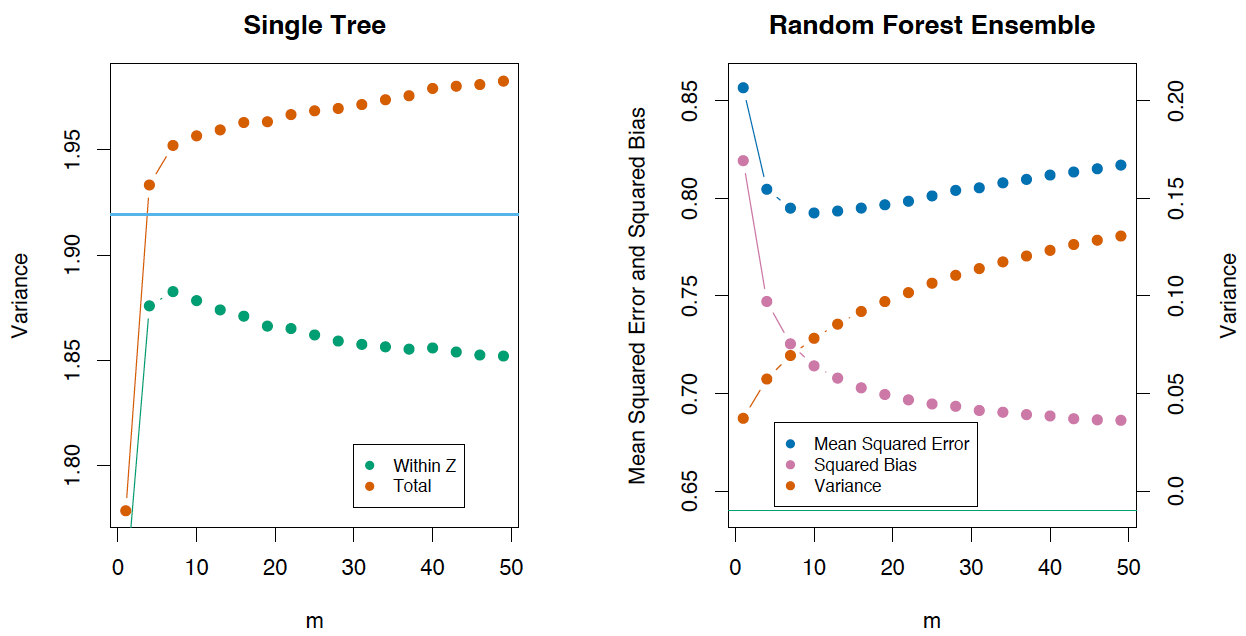#### 随机森林的偏差¶

$$\begin{array}{rcl} \mathrm{Bias} &=& \mu(x) - \mathrm{E}_Z \, f_{rf}(x) \\ &=& \mu(x) - \mathrm{E}_Z \, \mathrm{E}_{\Theta | Z} \, T(x,\Theta(Z))\end{array}$$

#### 极端随机树¶

scikit-learn 库的 ExtraTreesClassifier()ExtraTreesRegressor() 类实现了该方法。如果使用随机森林或梯度提升遇到了严重的过拟合，可以试试极端随机树。

#### 随机森林和 k 近邻的相似性¶

$$b_n(x) = \sum_{i=1}^{l}w_n(x,x_i)y_i,$$

$$w_n(x, x_i) = \frac{\left[T_n(x) = T_n(x_i)\right]}{\sum_{j=1}^{l}\left[T_n(x) = T_n(x_j)\right]}$$

$$\begin{array}{rcl} a_n(x) &=& \frac{1}{N}\sum_{n=1}^{N}\sum_{i=1}^{l}w_n(x,x_i)y_i \\ &=& \sum_{i=1}^{l}\left(\frac{1}{N}\sum_{j=1}^{N}w_n(x,x_j)\right)y_i \end{array}$$

• 基于随机森林或梯度提升技术在样本上训练决策树的复合模型
• 再将类别特征 $T_1(x), \dots, T_n(x)$ 加入样本。

$T_1(x), \dots, T_n(x)$ 特征是非线性空间分割的结果，它们提供了关于样本之间的相似性的信息。《The Elements of Statistical Learning》一书中有一个例子，演示了随机森林和 k-近邻技术的相似性，如下图所示：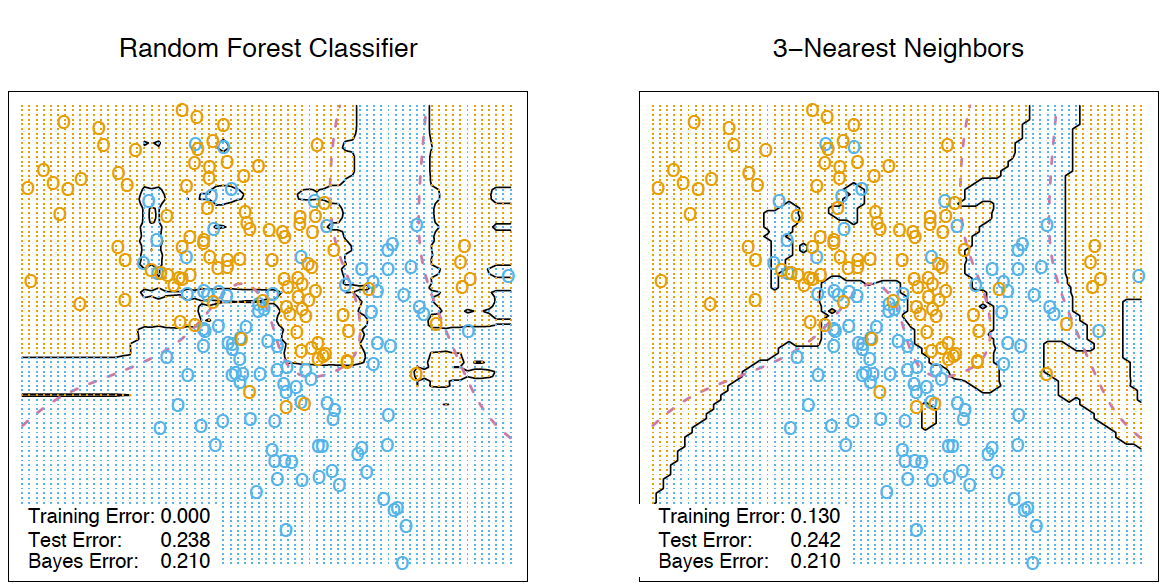#### 随机森林的优势和劣势¶

• 高预测准确率。在大多数问题上表现优于线性算法，准确率与 Boosting 相当；
• 随机取样导致其对离散值的鲁棒性较好；
• 随机选取子空间导致其对特征缩放及其他单调转换不敏感；
• 不需要精细的参数调整。
• 在具有大量特征和类别的数据集上很高效；
• 既可处理连续值，也可处理离散值；
• 不容易出现过拟合。在实践中，增加树的数量几乎总是能提升总体表现。不过，当树的数量增大到一定值后，学习曲线将趋于平稳；
• 有理论方法可以估计特征的重要性；
• 能够很好地处理数据缺失。即使有大部分的数据缺失现象，仍能保持较好的准确率；
• 支持整个数据集及单棵树样本上的加权分类；
• 决策树底层使用的相似性计算可以用于后续的聚类、离散值检测或兴趣数据表示；
• 以上功能和性质可以扩展到未标注数据，以支持无监督聚类，数据可视化和离散值检测；
• 易于并行化，伸缩性强。

• 相比单棵决策树，随机森林的输出更难解释；
• 特征重要性估计没有形式化的 p 值；
• 在数据稀疏时（比如，文本输入、词袋），表现不如线性模型好；
• 和线性回归不同，随机森林无法外推。不过，这样也就不会因为离散值的存在导致极端值的出现；
• 在某些问题上容易过拟合，特别是处理高噪声数据时；
• 处理数量级不同的类别数据时，随机森林偏重数量级较高的数据，因为这能更明显的提高准确率；
• 所得模型较大，需要大容量的 RAM 来支撑。

### 特征的重要性¶

#### 方法精要¶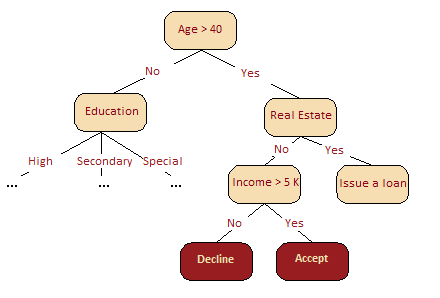$$VI^{T} = \frac{\sum_{i \in \mathfrak{B}^T}I \Big(y_i=\hat{y}_i^{T}\Big)}{\Big |\mathfrak{B}^T\Big |} - \frac{\sum_{i \in \mathfrak{B}^T}I \Big(y_i=\hat{y}_{i,\pi_j}^{T}\Big)}{\Big |\mathfrak{B}^T\Big |}$$

• $\hat{y}_i^{(T)} = f^{T}(x_i)$ 是特征置换或排除之前的类型预测；
• $\hat{y}_{i,\pi_j}^{(T)} = f^{T}(x_{i,\pi_j})$ 是特征置换或排除后的类型预测;
• $x_{i,\pi_j} = (x_{i,1}, \dots , x_{i,j-1}, \quad x_{\pi_j(i),j}, \quad x_{i,j+1}, \dots , x_{i,p})$.
• 注意，若 $x_j$ 不在树 $T$ 中，那么 $VI^{T}(x_j) = 0$。

• 未经正则化时的公式：
$$VI(x_j) = \frac{\sum_{T=1}^{N}VI^{T}(x_j)}{N}$$
• 使用标准差正则化后的公式：
$$z_j = \frac{VI(x_j)}{\frac{\hat{\sigma}}{\sqrt{N}}}$$

#### 计算现实问题中的特征重要性¶

In :
from __future__ import division, print_function

import numpy as np
import pandas as pd
import seaborn as sns
from matplotlib import pyplot as plt
from sklearn.ensemble.forest import RandomForestRegressor

%matplotlib inline


In :
hostel_data = pd.read_csv(
"../../data/hostel_factors.csv")
features = {"f1": u"Staff",
"f2": u"Hostel booking",
"f3": u"Check-in and check-out",
"f4": u"Room condition",
"f5": u"Shared kitchen condition",
"f6": u"Shared space condition",
"f7": u"Extra services",
"f8": u"General conditions & conveniences",
"f9": u"Value for money",
"f10": u"Customer Co-creation"}


In :
forest = RandomForestRegressor(n_estimators=1000, max_features=10,
random_state=0)

forest.fit(hostel_data.drop(['hostel', 'rating'], axis=1),
hostel_data['rating'])
importances = forest.feature_importances_

indices = np.argsort(importances)[::-1]


In :
num_to_plot = 10
feature_indices = [ind+1 for ind in indices[:num_to_plot]]


In :
print("Feature ranking:")

for f in range(num_to_plot):
print("%d. %s %f " % (f + 1,
features["f"+str(feature_indices[f])],
importances[indices[f]]))
plt.figure(figsize=(15, 5))
plt.title(u"Feature Importance")
bars = plt.bar(range(num_to_plot),
importances[indices[:num_to_plot]],
color=([str(i/float(num_to_plot+1))
for i in range(num_to_plot)]),
align="center")
ticks = plt.xticks(range(num_to_plot),
feature_indices)
plt.xlim([-1, num_to_plot])
plt.legend(bars, [u''.join(features["f"+str(i)])
for i in feature_indices])

Feature ranking:
1. Staff 0.182757
2. Value for money 0.148373
3. Shared space condition 0.128296
4. Extra services 0.116604
5. Customer Co-creation 0.106668
6. General conditions & conveniences 0.088589
7. Shared kitchen condition 0.074273
8. Check-in and check-out 0.061521
9. Hostel booking 0.053615
10. Room condition 0.039305

Out:
<matplotlib.legend.Legend at 0x7febd926ad68>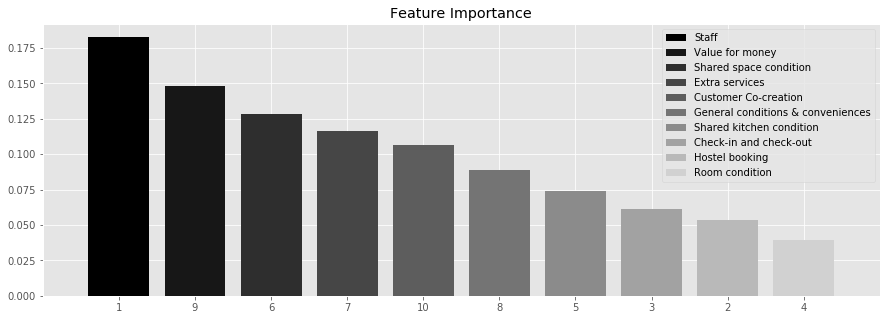#### 梯度提升介绍¶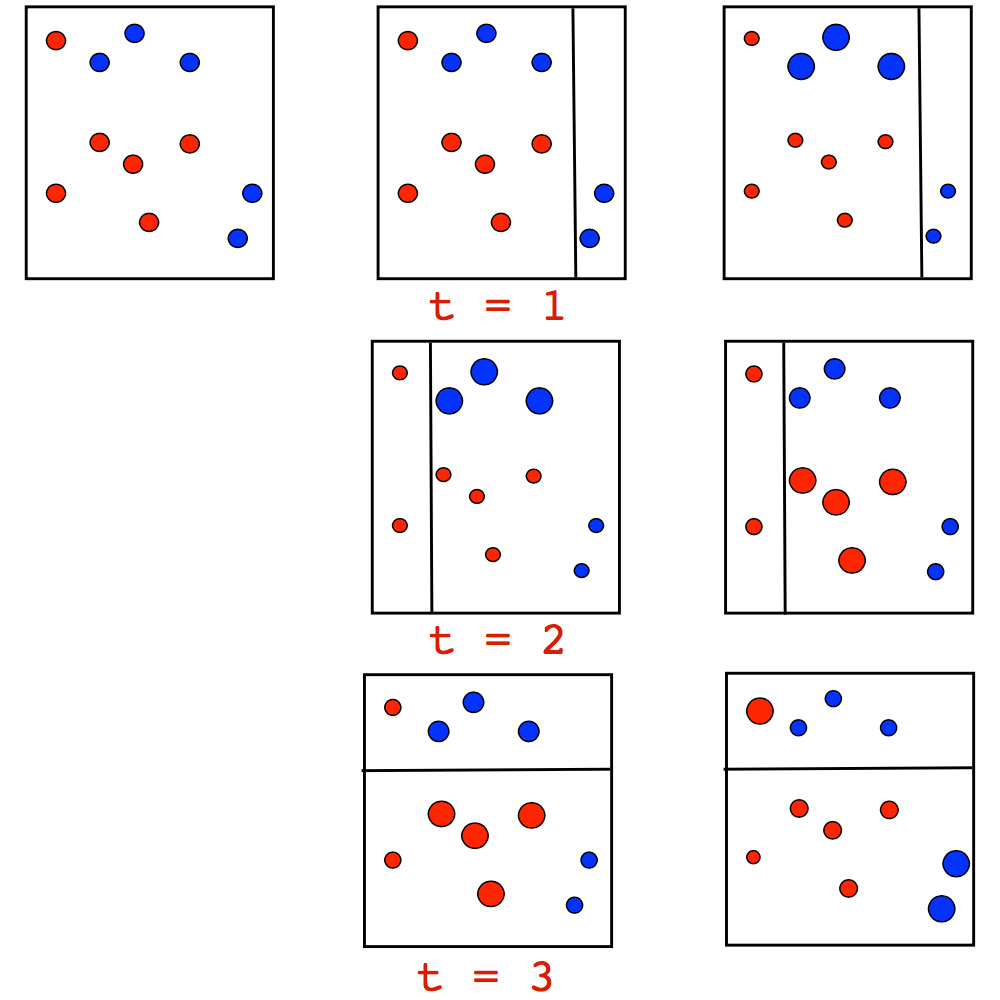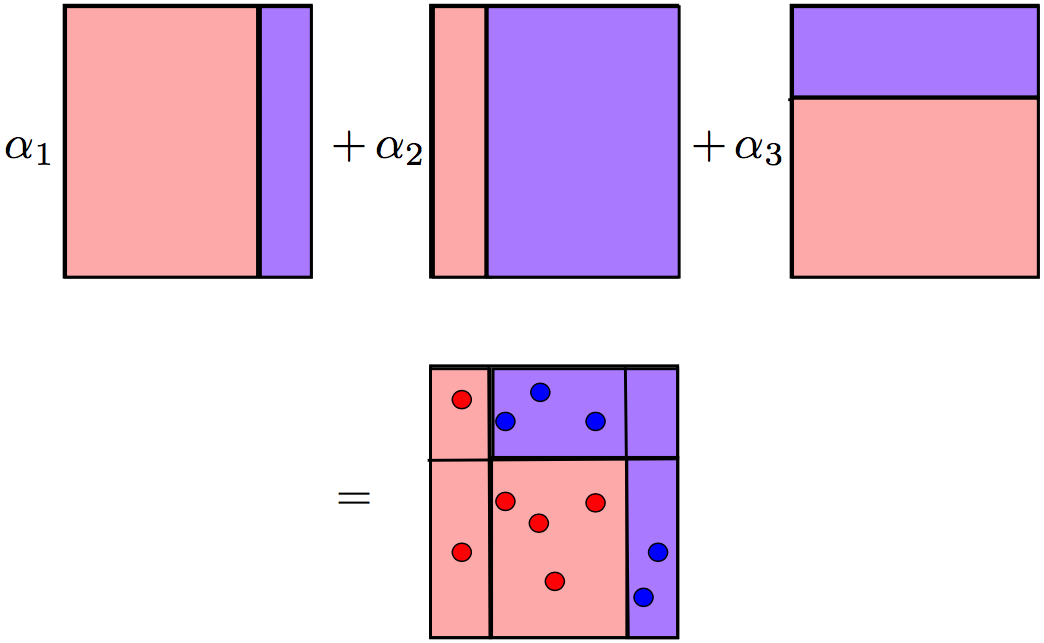• 首先，给每个样本一个初始的权重值：$w_i^{(0)} = \frac{1}{l}, i = 1, \dots, l$
• 遍历所有 $t = 1, \dots, T$
• 训练基分类器 $b_t$, 并令 $\epsilon_t$ 等于分类误差。
• $\alpha_t = \frac{1}{2}ln\frac{1 - \epsilon_t}{\epsilon_t}$
• 更新样本权重: $w_i^{(t)} = w_i^{(t-1)} e^{-\alpha_t y_i b_t(x_i)}, i = 1, \dots, l$
• 归一化样本权重: $w_0^{(t)} = \sum_{j = 1}^k w_j^{(t)}, w_i^{(t)} = \frac{w_i^{(t)}}{w_0^{(t)}}, i = 1, \dots, l$
• 返回 $\sum_t^{T}\alpha_tb_t$

CART、 bootstrap 和许多其他算法都起源于斯坦福大学的统计部门。这些算法都非常的实用，但也有一些近年来研究工作尚未得到广泛的应用。例如，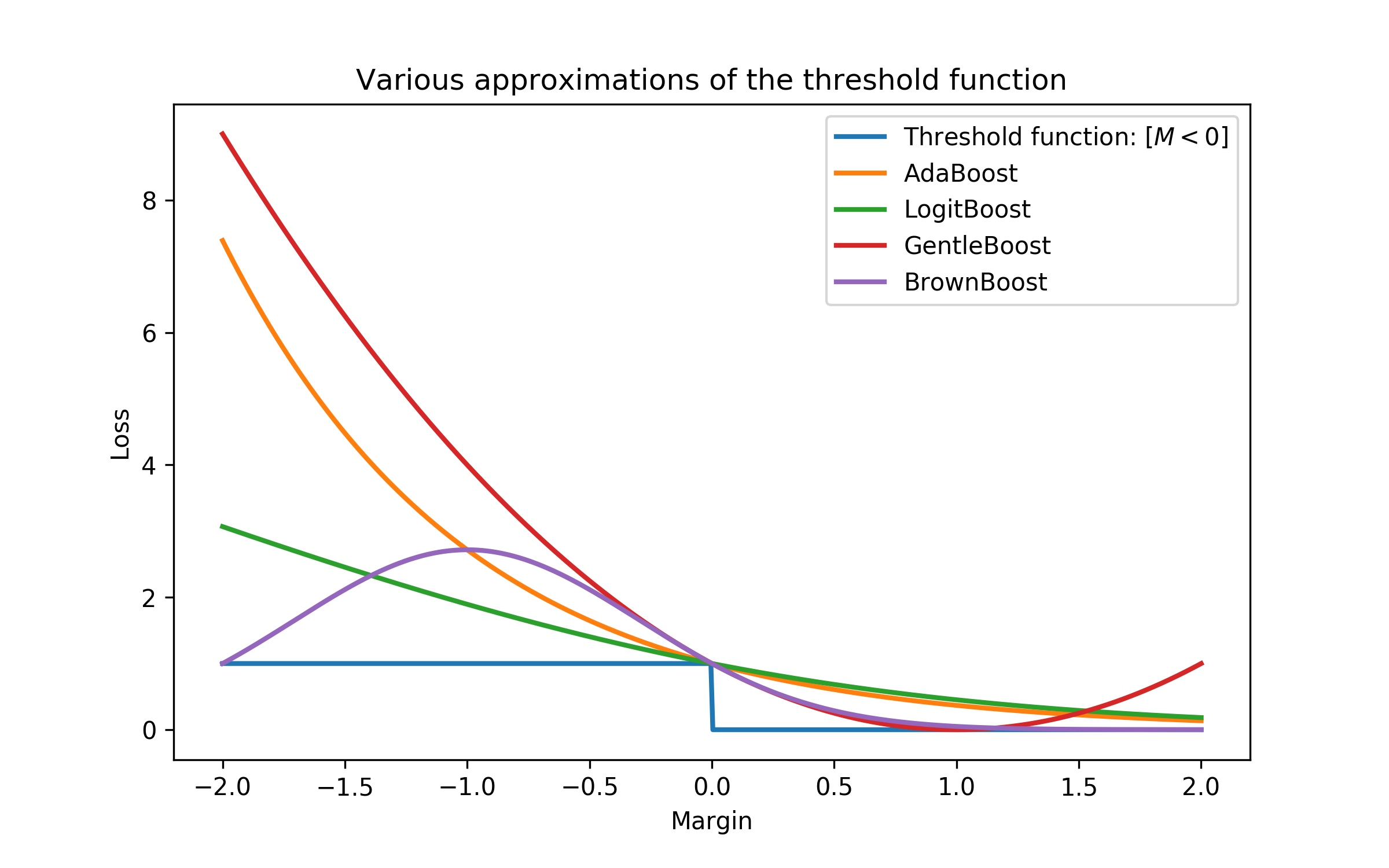#### GBM 的历史¶

GBM 的许多实现也以不同的名称出现在不同的平台上。例如：随机 GBM、GBDT (梯度提升决策树)、GBRT (梯度提升回归树)、MART (多元加性回归树)等等。此外，由于 ML 社区又是各自为营，所以这使我们很难去知道 GBM 到底得到多广泛的应用，到底有多少个版本等问题。GBM 的经历与许多机器学习算法一样：从被提出到成功的实践应用和大规模使用都花了许多年的时间。

### GBM 算法¶

$$y \approx \hat{f}(x)$$
$$\hat{f}(x) = \underset{f(x)}{\arg\min} \ L(y,f(x))$$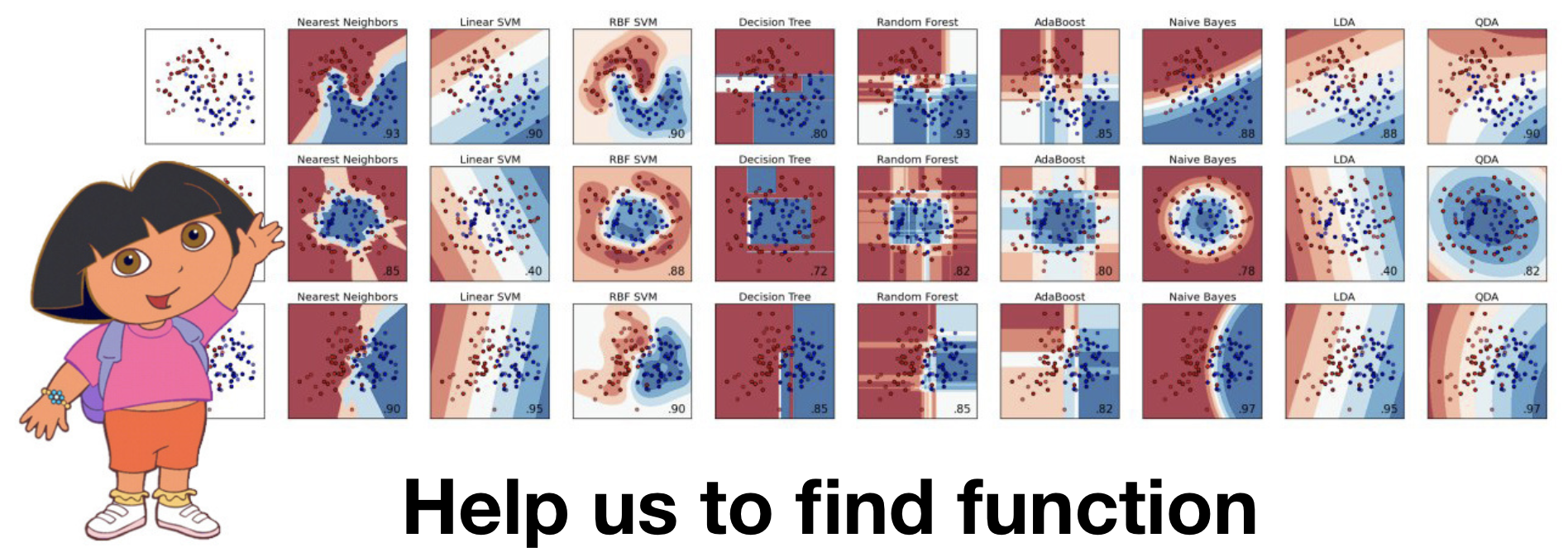$$\hat{f}(x) = \underset{f(x)}{\arg\min} \ \mathbb {E} _{x,y}[L(y,f(x))]$$

$$\hat{f}(x) = f(x, \hat{\theta})$$
$$\hat{\theta} = \underset{\theta}{\arg\min} \ \mathbb {E} _{x,y}[L(y,f(x,\theta))]$$

$$\hat{\theta} = \sum_{i = 1}^M \hat{\theta_i}$$
$$L_{\theta}(\hat{\theta}) = \sum_{i = 1}^N L(y_i,f(x_i, \hat{\theta}))$$

1. 定义参数的初始值 $\hat{\theta} = \hat{\theta}_0$
2. 对于每次迭代 $t = 1, \dots, M$ 重复步骤 3 到 7 :
3. 为当前的近似 $\hat{\theta}$ 计算损失函数的梯度值 $\nabla L_{\theta}(\hat{\theta}) = \left[\frac{\partial L(y, f(x, \theta))}{\partial \theta}\right]_{\theta = \hat{\theta}}$
4. 通过计算梯度来设置当前的近似 $\hat{\theta_t} \leftarrow −\nabla L_{\theta}(\hat{\theta})$
5. 更新参数 $\hat{\theta} \leftarrow \hat{\theta} + \hat{\theta_t} = \sum_{i = 0}^t \hat{\theta_i}$
6. 保存近似结果 $\hat{\theta} = \sum_{i = 0}^M \hat{\theta_i}$
7. 得到最终的函数 $\hat{f}(x) = f(x, \hat{\theta})$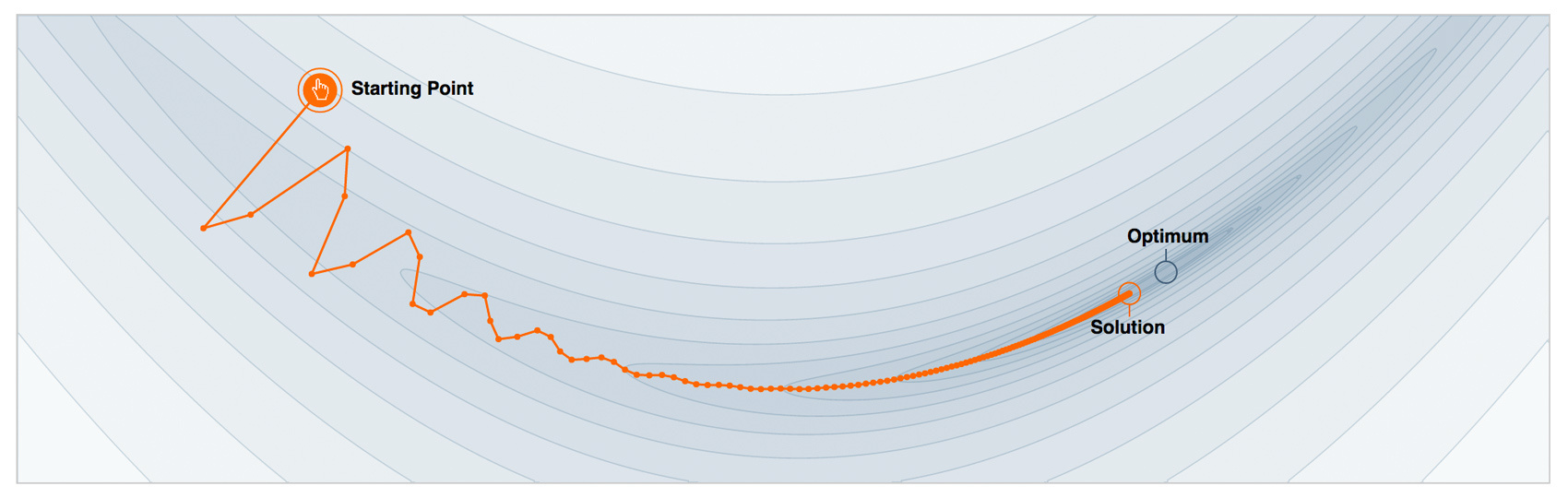#### 函数梯度下降¶

$$\hat{f}(x) = \sum_{i = 0}^M \hat{f_i}(x)$$

$$\hat{f}(x) = \sum_{i = 0}^{t-1} \hat{f_i}(x)$$
$$(\rho_t,\theta_t) = \underset{\rho,\theta}{\arg\min} \ \mathbb {E} _{x,y}[L(y,\hat{f}(x) + \rho \cdot h(x, \theta))]$$
$$\hat{f_t}(x) = \rho_t \cdot h(x, \theta_t)$$

$$\hat{f}(x) = \sum_{i = 0}^{t-1} \hat{f_i}(x)$$
$$r_{it} = -\left[\frac{\partial L(y_i, f(x_i))}{\partial f(x_i)}\right]_{f(x)=\hat{f}(x)}, \quad \mbox{for } i=1,\ldots,n$$
$$\theta_t = \underset{\theta}{\arg\min} \ \sum_{i = 1}^{n} (r_{it} - h(x_i, \theta))^2$$
$$\rho_t = \underset{\rho}{\arg\min} \ \sum_{i = 1}^{n} L(y_i, \hat{f}(x_i) + \rho \cdot h(x_i, \theta_t))$$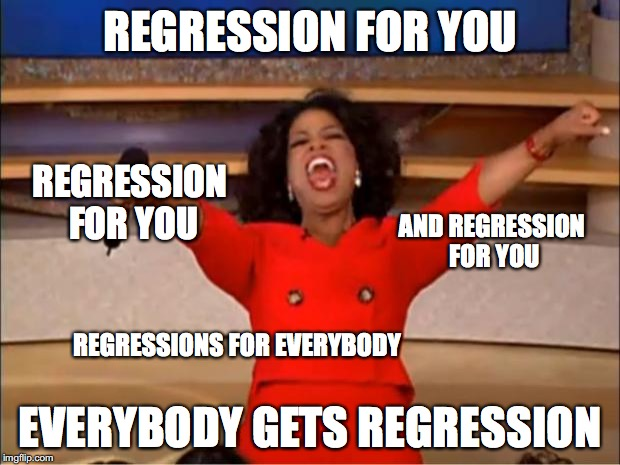#### Friedman 的经典 GBM 算法¶

• 数据集 $\left\{ (x_i, y_i) \right\}_{i=1, \ldots,n}$
• 迭代次数 $M$
• 损失函数 $L(y, f)$
• 函数空间 $h(x, \theta)$ 以及训练过程。
• 函数空间 $h(x, \theta)$ 的超参数。

1. 使用常数值初始化 GBM $\hat{f}(x) = \hat{f}_0, \hat{f}_0 = \gamma, \gamma \in \mathbb{R}$，$\hat{f}_0 = \underset{\gamma}{\arg\min} \ \sum_{i = 1}^{n} L(y_i, \gamma)$
2. 对于每次迭代 $t = 1, \dots, M$，重复逼近过程。
3. 计算伪残查 $r_t$，$r_{it} = -\left[\frac{\partial L(y_i, f(x_i))}{\partial f(x_i)}\right]_{f(x)=\hat{f}(x)}, \quad \mbox{for } i=1,\ldots,n$
4. 通过伪残差 $\left\{ (x_i, r_{it}) \right\}_{i=1, \ldots,n}$ 构建基算法$h_t(x)$
5. 通过 $h_t(x)$ 寻找最优系数。 $\rho_t = \underset{\rho}{\arg\min} \ \sum_{i = 1}^{n} L(y_i, \hat{f}(x_i) + \rho \cdot h(x_i, \theta))$
6. 保存 $\hat{f_t}(x) = \rho_t \cdot h_t(x)$
7. 更新 $\hat{f}(x) \leftarrow \hat{f}(x) + \hat{f_t}(x) = \sum_{i = 0}^{t} \hat{f_i}(x)$

8. 组成最终的模型 $\hat{f}(x) = \sum_{i = 0}^M \hat{f_i}(x)$

#### GBM 工作原理¶

$$y = cos(x) + \epsilon, \epsilon \sim \mathcal{N}(0, \frac{1}{5}), x \in [-5,5]$$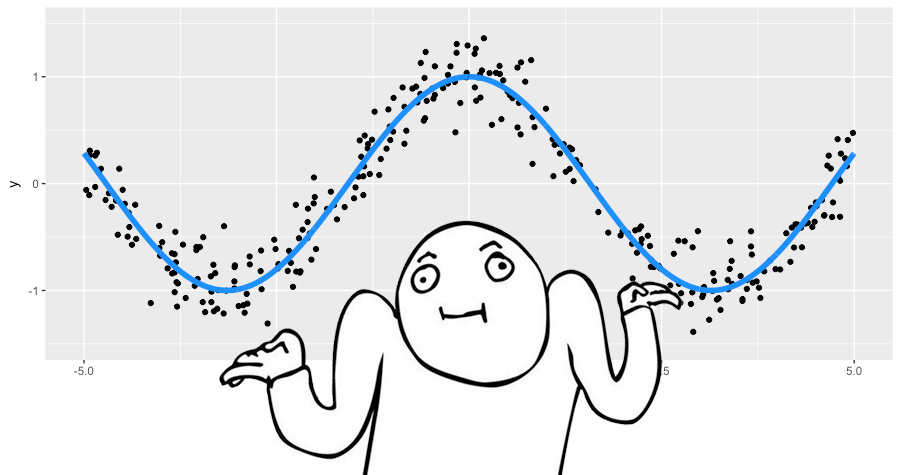• 数据集 $\left\{ (x_i, y_i) \right\}_{i=1, \ldots,300}$
• 迭代次数 $M = 3$
• 均方差损失函数 $L(y, f) = (y-f)^2$
• $L(y, f) = L_2$ 的梯度以及残差 $r = (y - f)$
• 基算法决策树 $h(x)$
• 决策树的超参数，树的深度为2。

$$\gamma = \frac{1}{n} \cdot \sum_{i = 1}^n y_i$$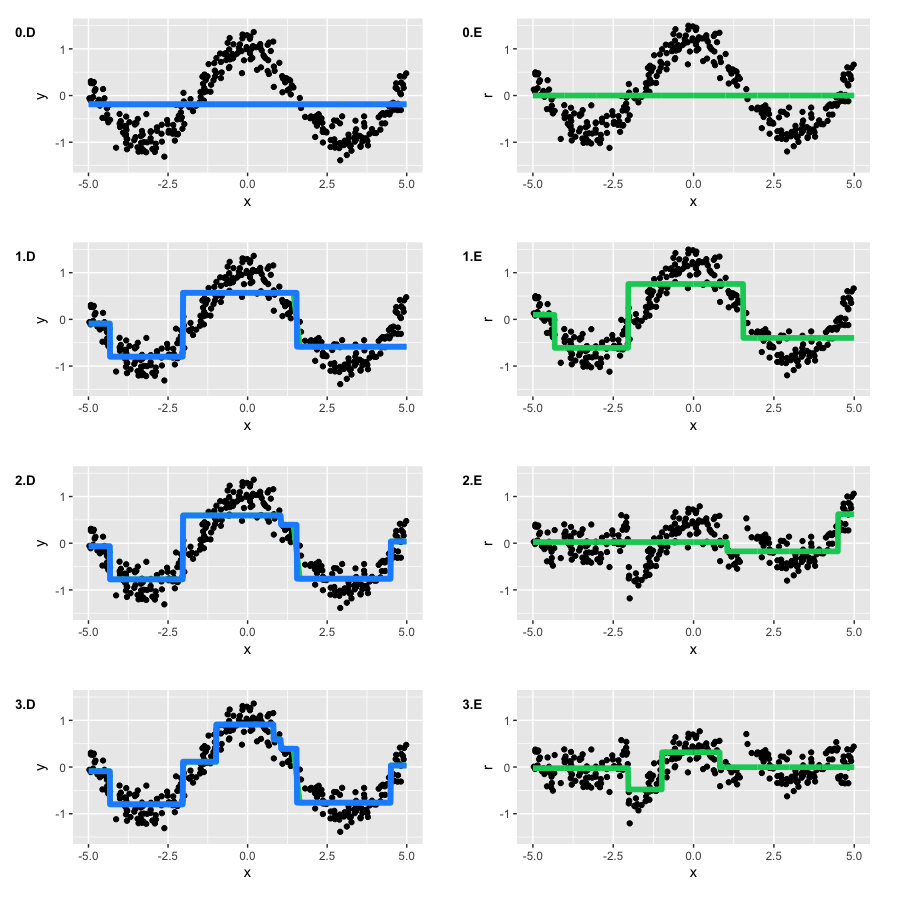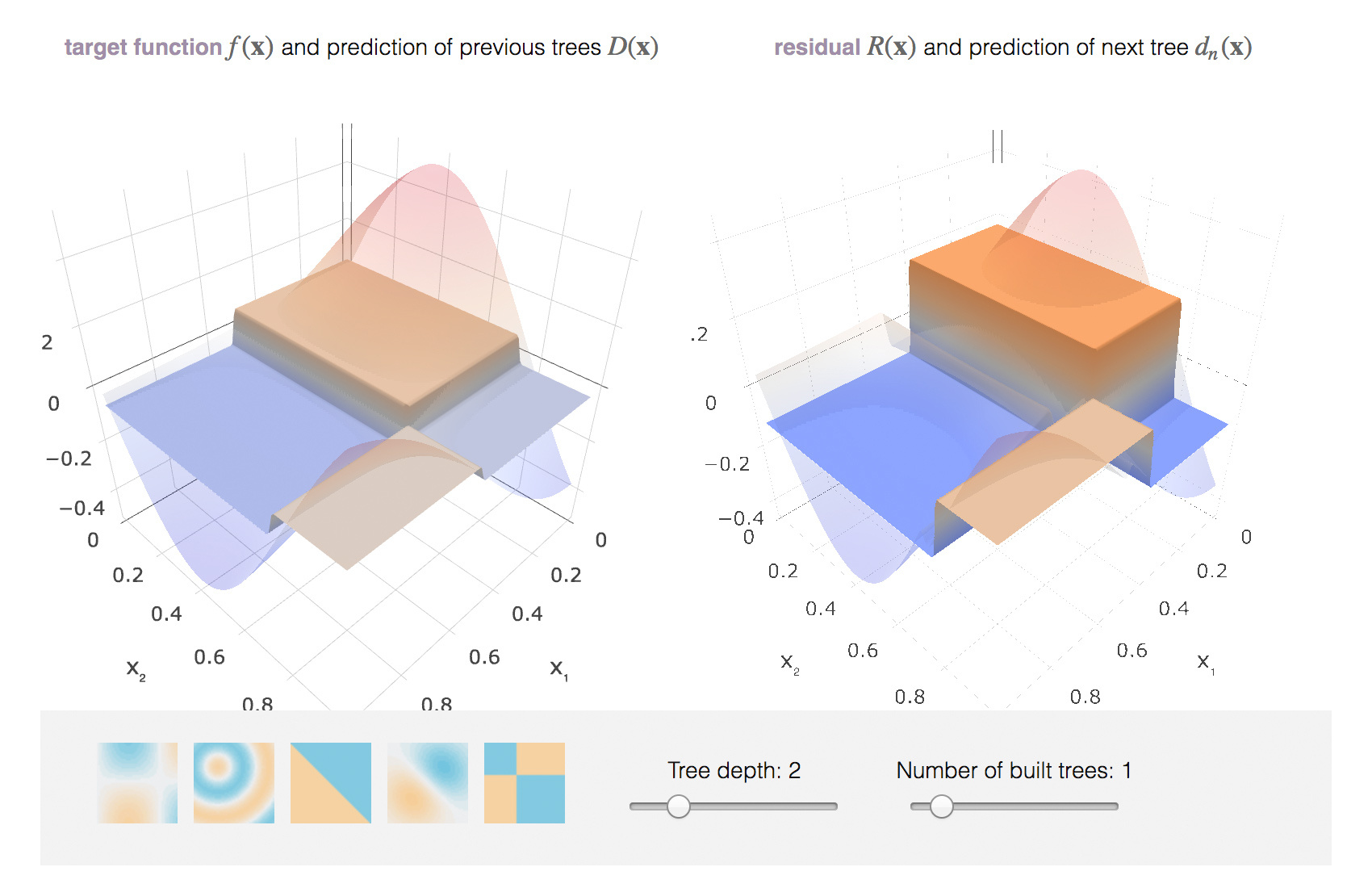### 损失函数¶

#### 回归损失函数¶

• $L_2$ 损失或高斯损失函数。该损失函数假定数据服从高斯分布。如果没有额外信息，也不要求模型的鲁棒性，那么可以使用该损失函数。公式如下：
$$L(y, f) = (y - f)^2$$
• $L_1$ 损失或拉普拉斯损失。事实上它定义了条件中位数。通常，中位数对离群值的鲁棒性更好，所以这一损失函数在某些情形下也表现更好。但对大偏差的惩罚不像 $L_2$ 那么大。公式如下：
$$L(y, f) = |y - f|$$
• $L_q$ 损失或分位数损失。与拉普拉斯损失相似， 只不过 $L_q$ 使用分位数而不是中位数。公式如下：
$$\begin{equation} L(y, f) =\left\{ \begin{array}{@{}[email protected]{}} (1 - \alpha) \cdot |y - f|, & \text{if}\ y-f \leq 0 \\ \alpha \cdot |y - f|, & \text{if}\ y-f >0 \end{array}\right. \end{equation}, \alpha \in (0,1)$$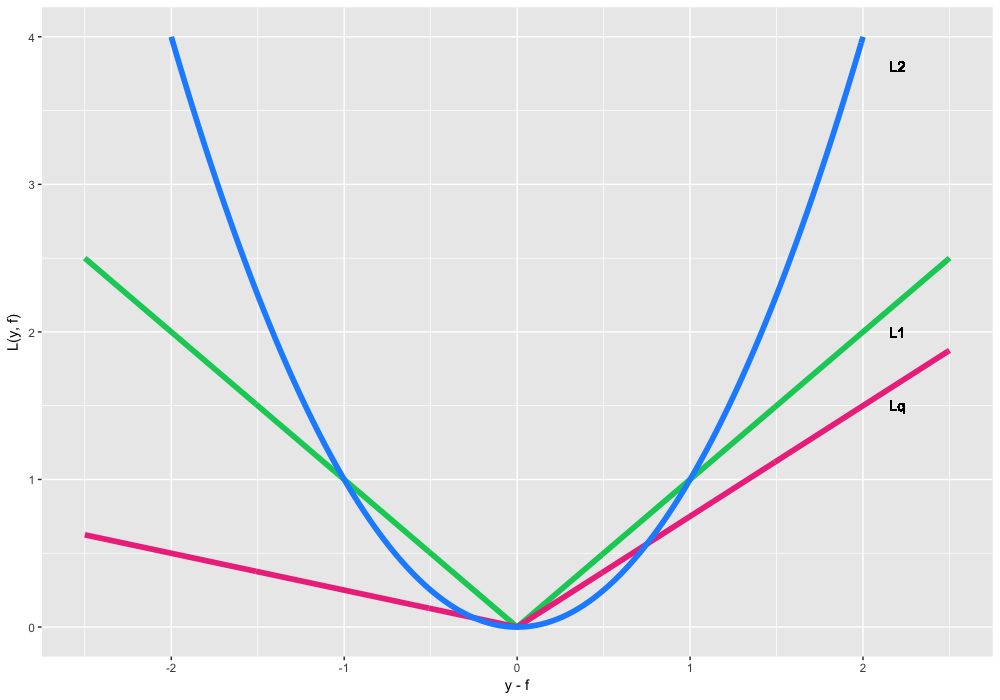• 数据 $\left\{ (x_i, y_i) \right\}_{i=1, \ldots,300}$
• 迭代次数 $M = 3$
• 损失函数 $\begin{equation} L_{0.75}(y, f) =\left\{ \begin{array}{@{}[email protected]{}} 0.25 \cdot |y - f|, & \text{if}\ y-f \leq 0 \\ 0.75 \cdot |y - f|, & \text{if}\ y-f >0 \end{array}\right. \end{equation}$
• 梯度 $L_{0.75}(y, f)$, $\alpha = 0.7$
• 基算法 $h(x)$ 为决策树。
• 决策树超参数：depth = 2。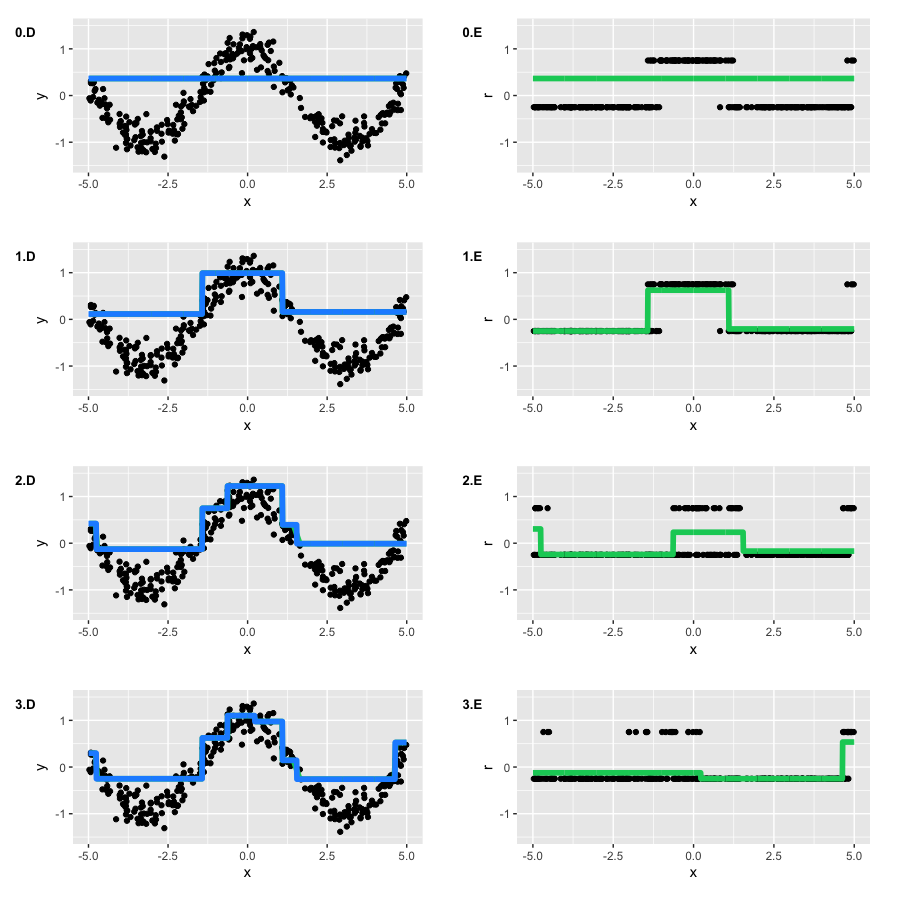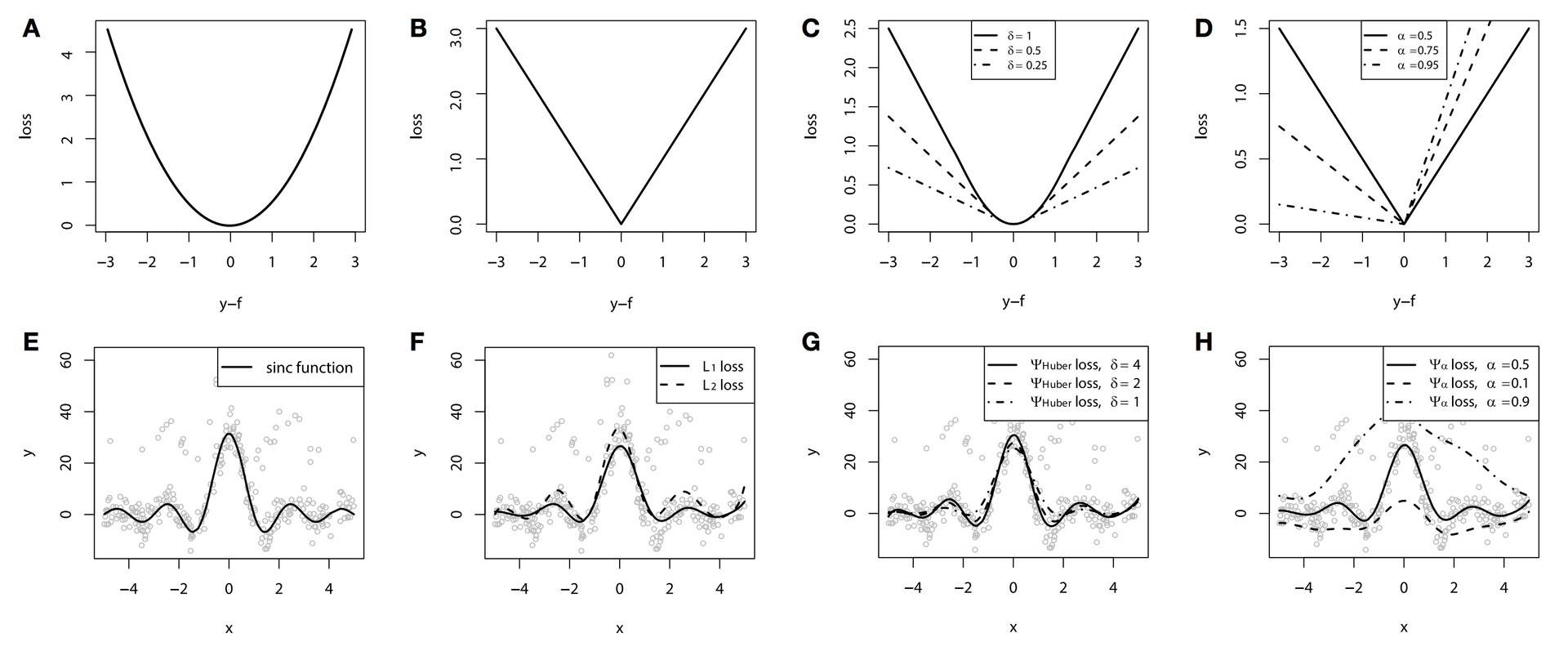#### 分类损失函数¶

• 逻辑损失或伯努利损失。该损失函数不仅要优化损失，同时要类别之间区别得更开。所以它甚至会惩罚正确预测的分类。公式如下：
$$L(y, f) = log(1 + exp(-2yf))$$

$$L(y, f) = exp(-yf)$$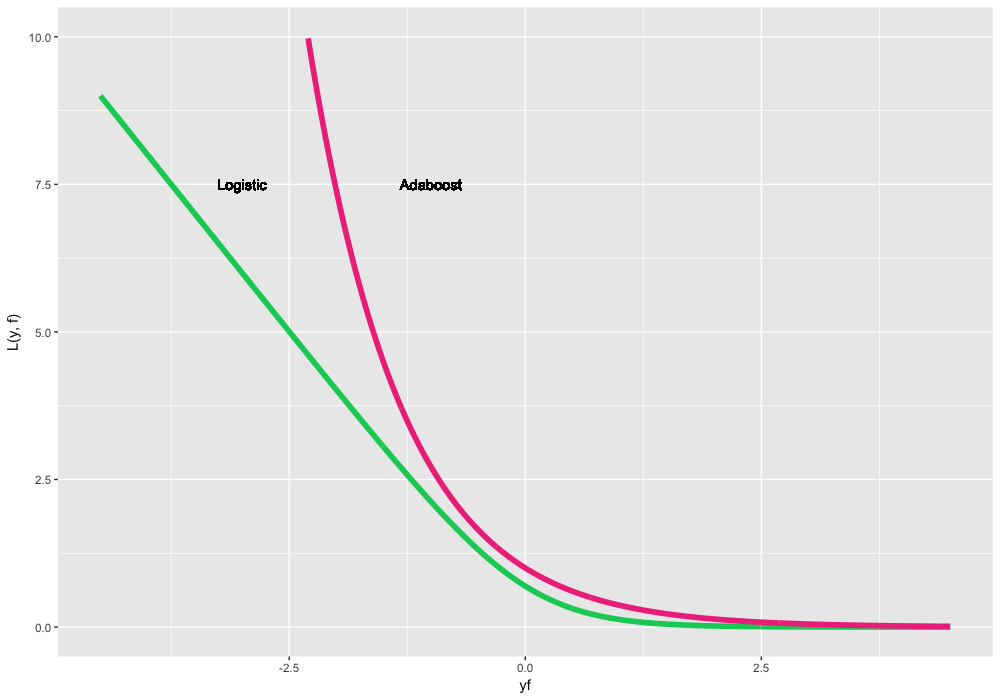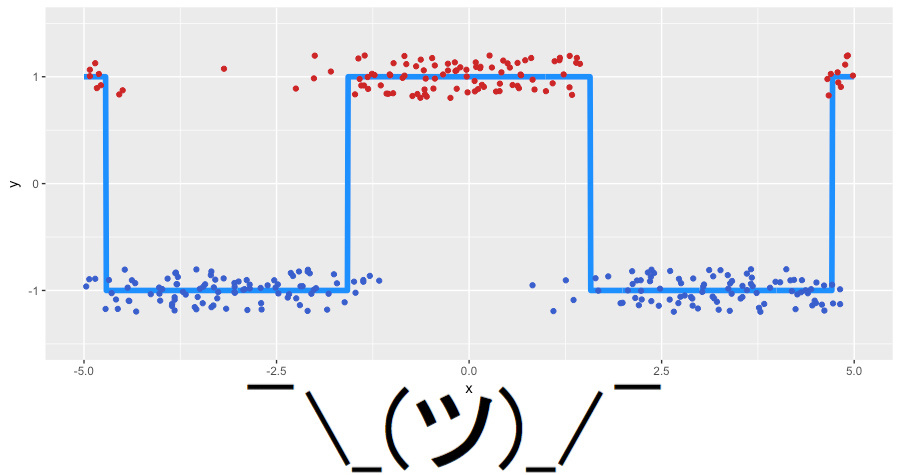• 实验数据：$\left\{ (x_i, y_i) \right\}_{i=1, \ldots,300}, y_i \in \left\{-1, 1\right\}$
• 迭代次数：$M = 3$
• 损失函数为逻辑损失函数, 其梯度为：$r_{i} = \frac{2 \cdot y_i}{1 + exp(2 \cdot y_i \cdot \hat{f}(x_i)) }, \quad \mbox{for } i=1,\ldots,300$
• 基算法为决策树：$h(x)$
• 决策树的超参数：depth = 2。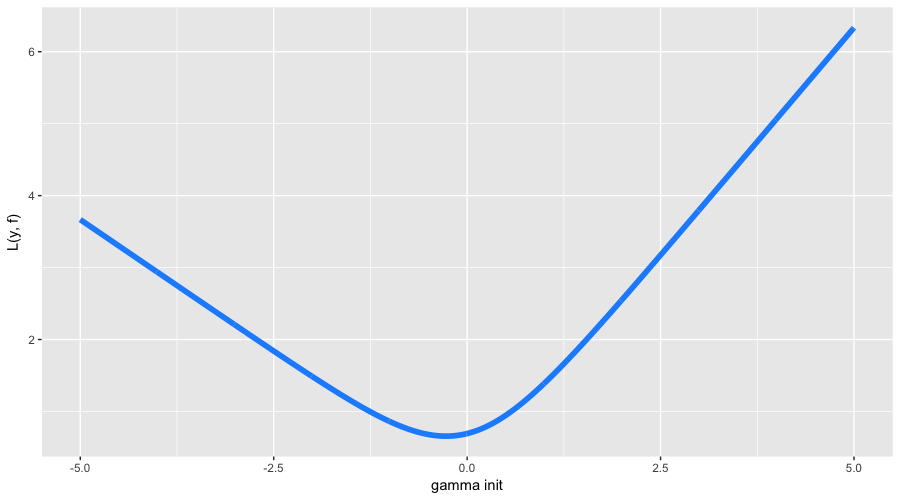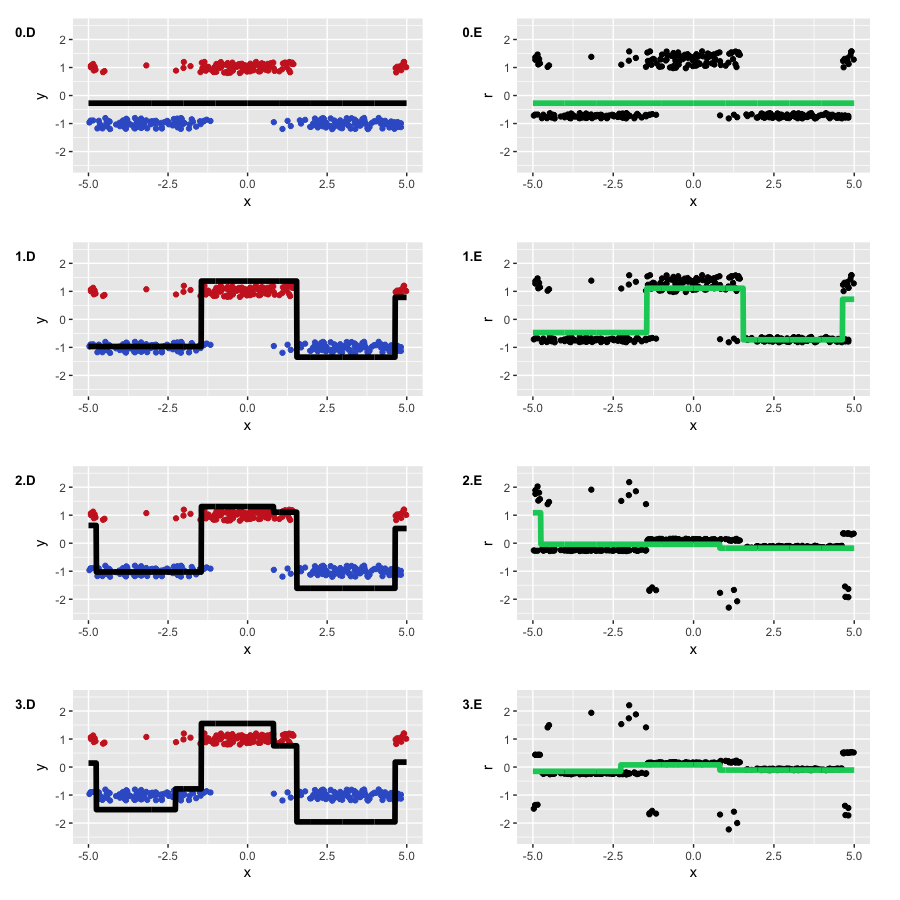#### 权重¶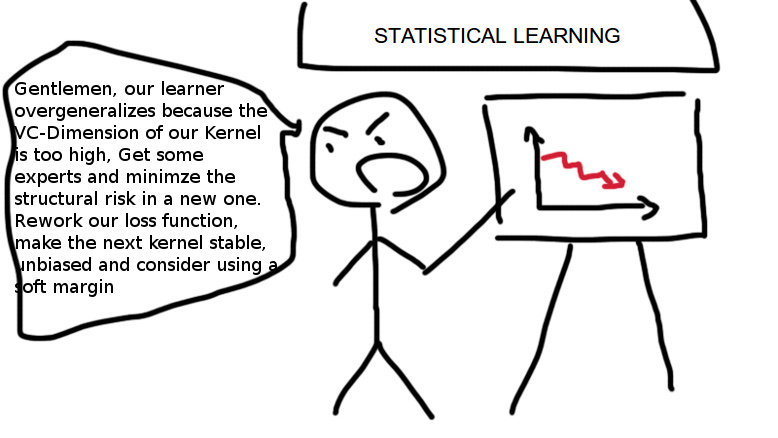$$w_i \in \mathbb{R},$$
$$w_i \geq 0 \quad \mbox{for } i=1,\ldots,n$$
$$\sum_{i = 1}^n w_i > 0$$

$$L_{w}(y,f) = w \cdot L(y,f)$$
$$r_{it} = - w_i \cdot \left[\frac{\partial L(y_i, f(x_i))}{\partial f(x_i)}\right]_{f(x)=\hat{f}(x)} \quad \mbox{for } i=1,\ldots,n$$

$$\begin{equation} w(x) =\left\{ \begin{array}{@{}[email protected]{}} 0.1, & \text{if}\ x \leq 0 \\ 0.1 + |cos(x)|, & \text{if}\ x >0 \end{array}\right. \end{equation}$$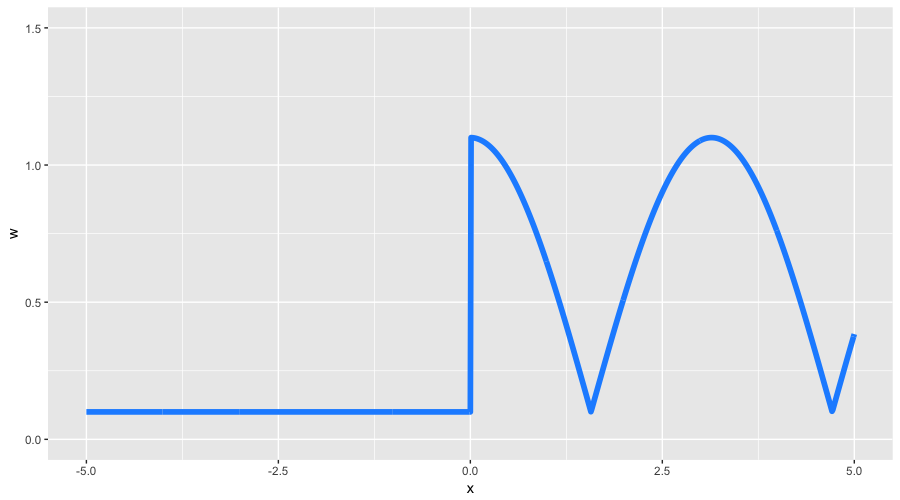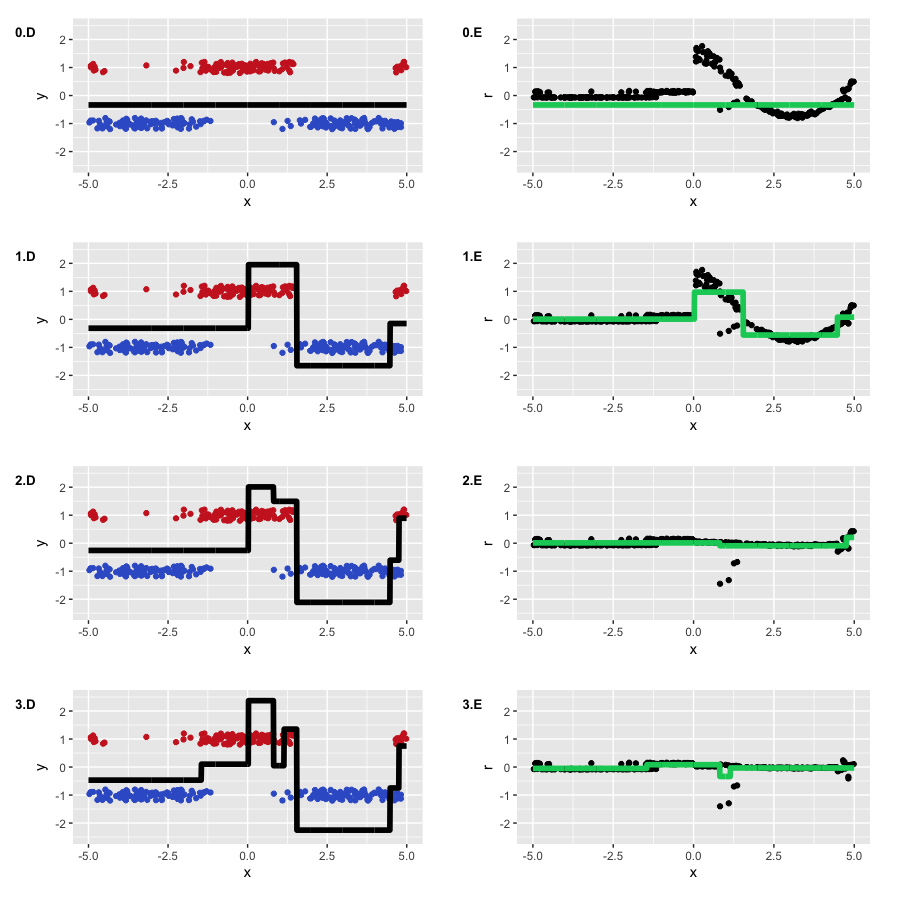#### 过拟合¶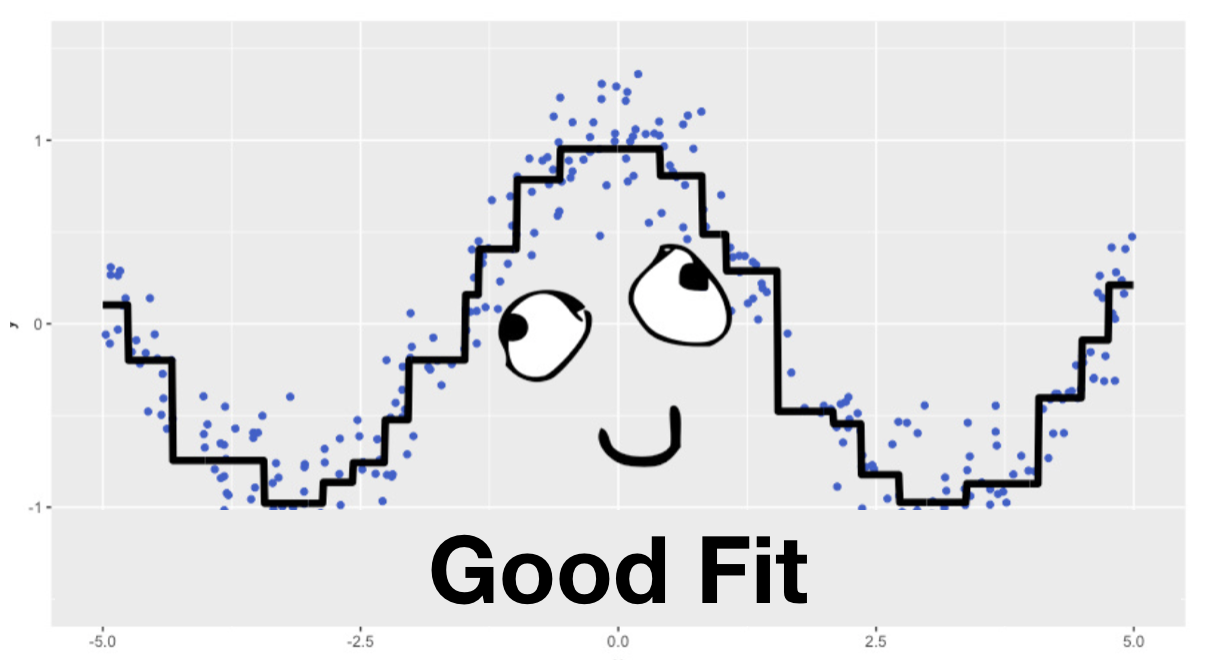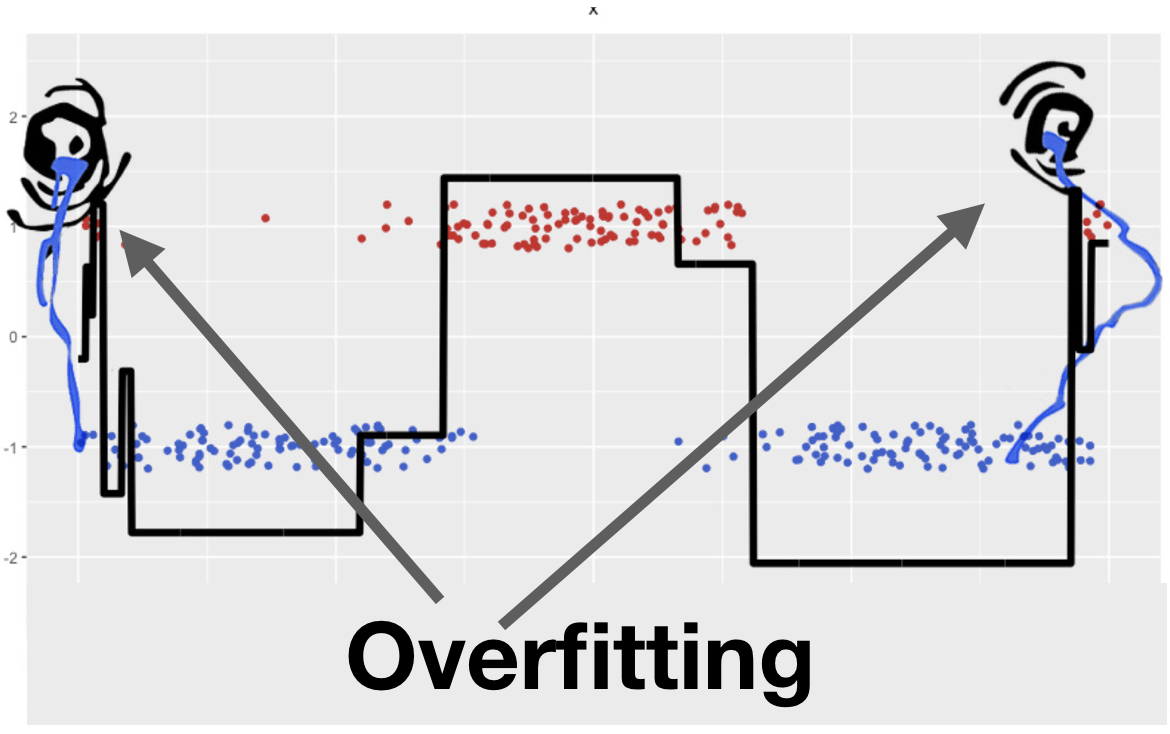30 棵决策树的拟合结果如下图所示，在训练集上得损失为 0 ，而在测试集上得损失为 0.664。这说明模型在训练集上过拟合了。